]]>
This code provides a implement for the linkedList in C. It offers create, transverse, sort, inversion, append, insert, del and other functions of the linkedList.

Please notice that the sort method actually can be replaced by some advanced algorithm. YOU can replace them manually. This code only offers the simplest way to sort for popular and easy to understand.

#include <stdio.h>
#include <malloc.h>
#include <stdlib.h>
#include <stdbool.h>

typedef struct Node {
int data;
struct Node * pNext;
} Node, *pNode;

pNode createList(int length);
bool insert(pNode pHead, int pos, int value);

int main(void) {
int length;

printf("Please input how many nodes do you want to make: ");
int len;
scanf("%d", &len);
printf("The minimum number is %d\n", minL(pHead));
printf("The maximum number is %d\n", maxL(pHead));

return 0;
}

pNode createList(int length) {
printf("Failed to allocate memory!");
exit(0);
}

for (int i = 0; i < length; i++) {
pNode ptr = (pNode)malloc(sizeof(Node));
if (ptr == NULL) {
printf("Failed to allocate memory!");
exit(0);
}
printf("Please input data of %d node: ", i + 1);
scanf("%d", &(ptr->data));
ptr->pNext = NULL;
*pTemp = ptr;
pTemp = &(ptr->pNext);
}
}

while (ptr->pNext != NULL) {
printf("%d ", ptr->pNext->data);
ptr = ptr->pNext;
}
printf("\n");
}

int len = 0;
while (pTemp->pNext != NULL) {
len++;
pTemp = pTemp->pNext;
}
return len;
}

while (pTemp != NULL) {
if (pTemp->data < min)
min = pTemp->data;
pTemp = pTemp->pNext;
}
return min;
}

while (pTemp != NULL) {
if (pTemp->data > max)
max = pTemp->data;
pTemp = pTemp->pNext;
}
return max;
}

bool append(pNode pHead, int value) {
pNode pTemp = (pNode)malloc(sizeof(Node));

if (pTemp == NULL) {
printf("Failed to allocate memory!");
exit(0);
}
pTemp->data = value;
pTemp->pNext = NULL;

while (ptr->pNext != NULL) {
ptr = ptr->pNext;
}
ptr->pNext = pTemp;

return true;
}

bool del(pNode pHead, int pos) {
if (pos < 1 || pos > getLength(pHead)) {
printf("Index out of bounds");
exit(0);
}
for (int i = 0; i < pos - 1; i++) {
ptr = ptr->pNext;
}

pNode r = ptr->pNext;
ptr->pNext = ptr->pNext->pNext;
free(r);

return true;
}

bool insert(pNode pHead, int pos, int value) {
if (pos < 1 || pos > getLength(pHead) + 1) {
printf("Index out of bounds");
exit(0);
}

for (int i = 0; i < pos - 1; i++) {
ptr = ptr->pNext;
}
pNode pTemp = ptr->pNext;

ptr->pNext = (pNode)malloc(sizeof(Node));
if (ptr->pNext == NULL) {
printf("Failed to allocate memory!");
exit(0);
}

ptr->pNext->data = value;
ptr->pNext->pNext = pTemp;

return true;
}

int get(pNode pHead, int pos) {
if (pos < 1 || pos > getLength(pHead)) {
printf("Index out of bounds");
exit(0);
}

for (int i = 0; i < pos; i++) {
ptr = ptr->pNext;
}

return ptr->data;
}

pNode ptr;
int min;
pNode pos;

for (int i = 0; i < len; i++) {
for (int m = 0; m < i; m++)
ptr = ptr->pNext;
min = ptr->data;
pos = ptr;
for (int k = i; k < len; k++) {
if (ptr->data < min) {
min = ptr->data;
pos = ptr;
}
ptr = ptr->pNext;
}
for (int m = 0; m < i; m++)
ptr = ptr->pNext;
pos->data = ptr->data;
ptr->data = min;
}
}

pNode a;
pNode ptr;
int temp;

for (int i = 0; i < len / 2; i++) {
for (int m = 0; m < i; m++)
ptr = ptr->pNext;
a = ptr;
for (int k = i; k < len - i - 1; k++)
ptr = ptr->pNext;
temp = ptr->data;
ptr->data = a->data;
a->data = temp;
}
}

]]>
<![CDATA[EJS - 1: Using EJS to show warning in expired article]]> https://blog.blinkstar.cn/post/ejs-date 2020-01-24T03:37:09.000Z This article shows how to use EJS code to show warning in expired article.

]]>
This article shows how to use EJS code to show warning in expired article.

First, put this ejs code in your ejs file at an appropriate place:

<%- outputDate();%><%  -%>
<%
function refineContent(str){
return str.replace("-","/");
}
function outputDate(){
let pDate= post.dateFormat;
pDate = refineContent(pDate);
var date2=new Date();
var date3=date2.getTime()-new Date(pDate).getTime();
var days=Math.floor(date3/(24*3600*1000));
if(days>=365){
content='<div class="warn"style="text-align:center">本文已超过 1 年没有更新，涉及的内容可能已经失效！</div>';
return content;
}
}


Then, using following css in your website:

.warn{
overflow:hidden;
margin:10px 0;
border:1px solid #eac946;
background:#fff4b9 -1px -1px no-repeat;
font-size:14px
}


Renderings:

]]>
<![CDATA[Data Structure - ArrayList]]> https://blog.blinkstar.cn/post/data-structure-arraylist 2020-01-19T03:42:01.000Z ArraList in C. The CODE implements the following function: Create an ArrayList; Display an ArrayList; Inverse an ArrayList; Sort an ArrayList; Get a value in ArrayList; Get Maximum and Minimum value in ArrayList; Append / Insert / Delete value in ArrayList.

]]>
ArraList in C. The CODE implements the following function: Create an ArrayList; Display an ArrayList; Inverse an ArrayList; Sort an ArrayList; Get a value in ArrayList; Get Maximum and Minimum value in ArrayList; Append / Insert / Delete value in ArrayList.

#include <stdio.h>
#include <stdlib.h>
#include <malloc.h>
#include <stdbool.h>

#define arrayList aL

struct arrayList {
int * pBase;
int len;
int cnt;
};

void init(struct aL * arr, int length);
void show(struct aL arr);
void inversion(struct aL * arr);
void sort(struct aL * arr);
int get(struct aL arr, int pos);
int maxA(struct aL arr);
int minA(struct aL arr);
bool append(struct aL * arr, int value);
bool insert(struct aL * arr, int pos, int value);
bool del(struct aL * arr, int pos);
bool isEmpty(struct aL arr);
bool isFull(struct aL arr);

int main(void) {
struct aL array;
init(&array, 6);
append(&array, 1);
append(&array, 2);
append(&array, 3);
append(&array, 4);
append(&array, 5);
insert(&array, 6, -1);
del(&array, 2);
show(array);
printf("%d\n", get(array, 5));
inversion(&array);
show(array);
printf("%d\n", maxA(array));
sort(&array);
show(array);
return 0;
}

void init(struct aL * arr, int length) {
arr->pBase = (int *)malloc(sizeof(int) * length);
if (arr->pBase == NULL) {
printf("分配失败");
exit(-1);
}
arr->len = length;
arr->cnt = 0;
}

bool isEmpty(struct aL arr) {
if (arr.cnt == 0)
return true;
else
return false;
}

bool isFull(struct aL arr) {
if (arr.cnt == arr.len)
return true;
else
return false;
}

void show(struct aL arr) {
if (isEmpty(arr))
printf("NULL");
else {
for (int i = 0; i < arr.cnt; i++) {
printf("%d ", arr.pBase[i]);
}
printf("\n");
}
}

bool append(struct aL * arr, int value) {
if (isFull(*arr))
return false;
arr->pBase[arr->cnt] = value;
arr->cnt++;
return true;
}

bool insert(struct aL * arr, int pos, int value) {
if (isFull(*arr))
return false;
if (pos < 1 || pos > arr->cnt + 1)
return false;

arr->cnt++;

for (int k = arr->cnt - 1; k > pos - 1; k--) {
arr->pBase[k] = arr->pBase[k-1];
}
arr->pBase[pos - 1] = value;
return true;
}

bool del(struct aL * arr, int pos) {
if (isEmpty(*arr))
return false;
if (pos < 1 || pos > arr->cnt)
return false;

for (int k = pos - 1; k < arr->cnt - 1; k++) {
arr->pBase[k] = arr->pBase[k + 1];
}

arr->cnt--;
return true;
}

int get(struct aL arr, int pos) {
if (pos < 1 || pos > arr.cnt)
exit(-1);
else
return arr.pBase[pos - 1];
}

void inversion(struct aL * arr) {
int pre = 0, post = arr->cnt - 1, temp;

for (int i = 0; i < (arr->cnt / 2); i++) {
temp = arr->pBase[pre];
arr->pBase[pre] = arr->pBase[post];
arr->pBase[post] = temp;
post--;
pre++;
}
return true;
}

void sort(struct aL * arr) {
int t, pos;

for (int i = 0; i < arr->cnt; i++) {
t = arr->pBase[i];
pos = i;
for (int k = i; k < arr->cnt; k++){
if (arr->pBase[k] < t) {
pos = k;
t = arr->pBase[k];
}
}
arr->pBase[pos] = arr->pBase[i];
arr->pBase[i] = t;
}

return true;
}

int maxA(struct aL arr) {
int r = arr.pBase;

for (int i = 1; i < arr.cnt; i++) {
if (arr.pBase[i] > r)
r = arr.pBase[i];
}

return r;
}

int minA(struct aL arr) {
int r = arr.pBase;

for (int i = 1; i < arr.cnt; i++) {
if (arr.pBase[i] < r)
r = arr.pBase[i];
}

return r;
}

]]>

]]>

## Code

• Add the following css code in your css file(The idea of using mix-blend-mode comes from darkmode.js)
.darkmode-layer {
position: fixed;
pointer-events: none;
background: #fff;
mix-blend-mode: difference;
width: 100%;
height: 100%;
top: 0;
left: 0;
}
.darkmode-background {
background: #fff;
position: fixed;
pointer-events: none;
z-index: -1;
width: 100%;
height: 100%;
top: 0;
left: 0;
}
.darkmode .search-input {
background: #000;
border-bottom-color: inherit
}
.darkmode img {
mix-blend-mode: difference;
filter: grayscale(30%);
}
.darkmode pre {
background-color: #000 !important;
}
.darkmode .line-number {
color: #c1c1c1;
}
.darkmode .hidden_nav {
background-color: #000;
}
.switch_default[type=checkbox] {
display: none
}
.switch_default + label {
background-color: #e6e6e6;
cursor: pointer;
display: inline-block;
height: 14px;
position: relative;
box-shadow: .2px .2px 1px .5px #b4b4b4;
width: 30px
}
.switch_default + label:after {
background-color: #fff;
content: "";
height: 12px;
left: 1px;
position: absolute;
top: .5px;
width: 12px;
box-shadow: .2px .2px 1px .5px #b4b4b4
}
.switch_default:checked + label {
background-color: #1abc9c;
}
.switch_default:checked + label:after {
left: 17px
}

• Add the following html code to where you want to show the button to control the darkmode. (The design of this button comes from Chic)
<div><input id="switch_default" onclick="mobileBtn()" type="checkbox" class="switch_default"><label for="switch_default" class="toggleBtn"></label></div>


You can also write your own css to beautify this button.

• Put these two div beneath the body tag
<div id="darkmode_b"></div><div id="darkmode_l"></div>

• Add the following js beneath the div.
// Written by Serence: blog.blinkstar.cn
{
var arr,reg=new RegExp("(^| )"+name+"=([^;]*)(;|$)"); if(arr=document.cookie.match(reg)) return unescape(arr); else return null; } cookie = getCookie("darkmode"); if (cookie == "enable"){ document.getElementById("darkmode_b").classList.add("darkmode-background"); document.getElementById("darkmode_l").classList.add("darkmode-layer"); document.body.classList.add("darkmode"); document.getElementById("switch_default").checked = true; } var mobileBtn = function f() { if (document.getElementById("switch_default").checked == true){ document.getElementById("darkmode_b").classList.add("darkmode-background"); document.getElementById("darkmode_l").classList.add("darkmode-layer"); document.body.classList.add("darkmode"); document.cookie="darkmode=enable; path=/"; } else{ document.getElementById("darkmode_b").classList.remove("darkmode-background"); document.getElementById("darkmode_l").classList.remove("darkmode-layer"); document.body.classList.remove("darkmode"); document.cookie="darkmode=disable; path=/"; } }  you can also the compressed js. function getCookie(a){var b,c=new RegExp("(^| )"+a+"=([^;]*)(;|$)");return(b=document.cookie.match(c))?unescape(b):null}cookie=getCookie("darkmode"),"enable"==cookie&&(document.getElementById("darkmode_b").classList.add("darkmode-background"),document.getElementById("darkmode_l").classList.add("darkmode-layer"),document.body.classList.add("darkmode"),document.getElementById("switch_default").checked=!0);var mobileBtn=function(){1==document.getElementById("switch_default").checked?(document.getElementById("darkmode_b").classList.add("darkmode-background"),document.getElementById("darkmode_l").classList.add("darkmode-layer"),document.body.classList.add("darkmode"),document.cookie="darkmode=enable; path=/"):(document.getElementById("darkmode_b").classList.remove("darkmode-background"),document.getElementById("darkmode_l").classList.remove("darkmode-layer"),document.body.classList.remove("darkmode"),document.cookie="darkmode=disable; path=/")};


## Problems

you may notice that some of your picture may become very strange after using this way. Though I add .darkmode img to overwrite some of the img, it may still have some problems. You can try to use the following code to avoid any of your code block being covered by the new layer.

// This code uses a class named logo as an example
.darkmode-layer .logo {
isolation: isolate;
mix-blend-mode: difference;
}

]]>

]]>
This passage compiles the procedures to use adsense and some major problems like "avaliable width = 0" problem that people face when using adsense.

## 2. Preparation

• A blog with some original articles

## 3. Application - From Yiyun's Blog

1. Apply for adsense - Click
3. Waiting for verification - will last about 2 and 3 days

## 4. Configuraiton

Auto ads is put in your website by using machine learning techniques from google. You can also customize the settings to determine whether to show an ad in a certain places.

From its name, we can know that its for websites that has feed(usually this type of ad is put at the home page). You can determine which place to put this ad, and you can also determine how many ads do you want to place in your feed. If you are using gridea, you can look for the following code to add In-feed ads in your feed page:

<% if (seq % 3 == 0) {%> <ins class="adsbygoogle" //seq is from <% posts.forEach(function(post, seq) { %>
style="display:block"
</script> <%} %>


This type of ad is used in article. The formal suggestion is to put this ad at the end of the second paragraph of the article. This ad can automatically detect your content and put suitable ads in your article.

Besides these ads, you can also choose the type of ads you want to show in your website and manually censor all the ads that has appeared in your site.

In this place, you can approve or block ads.

However, this procedure may take you a lot of time. Thus, you can go to the Content ads to determine whether you want certain type of ads shown in your website(Like the ads about sex).## 5. Problems

But you will face some problems when using adsense.

• Fluid responsive ads must be at least 250px wide

There are two ways to solve this problem:

1. If you are using Jquery, you can use the following code from galeksic
<script>
/* Warning: this is non-recommended code */
});
</script>

1. I discover another solution to solve this problem:

Put the following css code in your css file:

ins{
width:100% !important;
display:block !important;
}

//Replace this code with the new one

//new
}


This can solve the problem, but sometimes it doesn't work either. But most of time, it can ensure that ads can be shown in your website.

Please notice: you can withdraw only when you earn greater than or equal 100in adsense. ]]> <![CDATA[2020 年度书单]]> https://blog.blinkstar.cn/post/2020-books 2020-01-09T15:37:02.000Z 这份书单集合了多种文学体裁，涵盖了反乌托邦、存在主义荒诞体裁、散文、科幻小说、历史、经济…… ]]> 这份书单集合了多种文学体裁，涵盖了反乌托邦、存在主义荒诞体裁、散文、科幻小说、历史、经济…… ## 概览 • Brave new world 美丽新世界 • The Stranger 局外人 • Walden 瓦尔登湖 • 基地7部曲 • A Brief History of Tomorrow 未来简史 • 国富论 • 巴黎圣母院 • 追风筝的人 • The Chrysanthemum and The Sword 菊与刀 ## 详情 • Brave new world 美丽新世界 一本极好的乌托邦小说，目前已经✅。作者以一种讽刺的角度来探究了科技高度发达的社会的运行情况，借野人这一角色反映了“美丽新世界”的冷血。 • The Stranger 局外人 一本很好的存在主义荒诞小说，目前已经✅。 • Walden 瓦尔登湖 一本很想看的散文书籍，作者记录了自己在瓦尔登湖的所见所闻所感。了解到这本书是因为死亡诗社，里面有一句话： I went to the woods because I wished to live deliberately, to front only the essential facts of life, and see if I could not learn what it had to teach, and not, when I came to die, discover that I had not lived. • 基地7部曲 非常好的科幻小说，很早之前就想看了，但是一直没有时间。 • Homo Deus: A Brief History of Tomorrow 未来简史 • 国富论 亚当斯密的国富论算是现代自由贸易理论的先驱，也是我非常想要了解的一个内容。 • 巴黎圣母院 去年巴黎圣母院着火牵动了无数人的心，正好我也没怎么看过雨果的书，所以就放到书单里了。 • 追风筝的人 • 菊与刀 了解日本文化的一部很好的作品。 ]]> <![CDATA[Wechat article pictures downloader]]> https://blog.blinkstar.cn/post/wechat-article-picture-downloader 2020-01-05T04:59:04.000Z This downloader can be used to download pictures from wechat article. ]]> This downloader can be used to download pictures from wechat article. # Preparation • Install reportlab and PyPDF2 in python (if you want to convert pictures into pdf) # Code import re import requests import os from os import remove,listdir from os.path import join from reportlab.lib.pagesizes import A4,landscape,portrait from reportlab.pdfgen import canvas from PyPDF2 import PdfFileReader,PdfFileMerger header={"user-agent":"Mozilla/5.0 (Windows NT 10.0; WOW64) AppleWebKit/537.36 (KHTML, like Gecko) Chrome/69.0.3464.0 Safari/537.36"} l = input("请输入下载链接:") daohang = requests.get(l,headers=header) daohang.encoding = ('utf8') da = re.findall('data-src="(.*?)"',daohang.text,re.S) path = re.findall('<h2 class="rich_media_title" id="activity-name">(.*?)</h2>',daohang.text,re.S) path = path.strip() print("正在下载中:") name = 1 for i in range(0,len(da)): print("正在下载第" + str(i) + "张图片") r=requests.get(da[i], headers=header) if not os.path.exists(path): os.makedirs(path) with open(path +"\\" + str(name) + ".jpeg",'wb') as code: code.write(r.content) code.close() name += 1 print("下载完成!\n") judge = input("是否需要转成pdf：是按1，不是按任意键退出：") if judge == "1": jpg_files = [path + "\\" + str(i)+".jpeg" for i in range(1,name)] result_pdf = PdfFileMerger() for fn in jpg_files: c = canvas.Canvas("temp.pdf", pagesize=portrait(A4)) c.drawImage(fn, 0, 0,*portrait(A4)) c.save() with open("temp.pdf","rb")as fp: pdf_reader = PdfFileReader(fp) result_pdf.append(pdf_reader) result_pdf.write(path + "\\" + "result.pdf") result_pdf.close() remove("temp.pdf") print("转换成功！") else: exit(0)  ]]> <![CDATA[JS TOC Generator]]> https://blog.blinkstar.cn/post/js-toc-generator 2020-01-03T07:32:32.000Z Automatically generate the table of content of passage ]]> Automatically generate the table of content of passage In order to fast generate table of content of my passage, I try tocbot.js before. But it is entirely based on javascript but my website support jquery. Thus, I decide to turn to the jquery table of content generator. Then I find autoMenu.js. And I improve this code to make sure that it can be used almost in every website. # Preparation • Put the following label in your website .autoMenu{ position: fixed; bottom: 10%; right: 5%; z-index: 1; text-align: left; } .autoMenu ul{ line-height: 2; overflow-y: auto; background: #fff; -webkit-box-shadow:0 0 10px #CCC; -moz-box-shadow:0 0 10px #CCC; box-shadow:0 0 10px #CCC; } .autoMenu ul>li.sub{ padding-left: 20px; } .autoMenu ul>li>a{ color: #399c9c; } .autoMenu ul>li.active>a{ color: #ff5370; } .btn-box{ display: inline-block; width: 40px; height: 40px; text-decoration: none; position: relative; } .icon-plus-sign{ display: inline-block; width: 26px; height: 26px; border-radius: 50%; border:4px solid #91ba61; background: #91ba61; position: absolute; top:3px; left:3px; } .icon-plus-sign:before,.icon-plus-sign:after{ content: ''; display: inline-block; width: 3px; height: 20px; background: #fff; border-radius: 1px; position: absolute; top:3px; left:11.5px; } .icon-plus-sign:after{ -webkit-transform:rotate(90deg); } .icon-minus-sign{ display: inline-block; width: 26px; height: 26px; border-radius: 50%; border:4px solid #ff5370; background: #ff5370; position: absolute; top:3px; left:3px; } .icon-minus-sign:before{ content: ''; display: inline-block; width: 20px; height: 3px; background: #fff; border-radius: 1px; position: absolute; left:3px; top:11.5px; }  • Then add the following div after your passage <div class="autoMenu" id="autoMenu" data-autoMenu> </div>  # Including js • Include this js in your passage page /* * blogMenu plugin 1.0 2017-09-01 by cary * 说明：自动根据标签（h3,h4）生成博客目录 */ (function () {

var Menu = (function () {
/**
* 插件实例化部分，初始化时调用的代码可以放这里
* @param element 传入jq对象的选择器，如 $("#J_plugin").plugin() ,其中$("#J_plugin") 即是 element
* @param options 插件的一些参数神马的
* @constructor
*/
var Plugin = function(element, options) {
//将dom jquery对象赋值给插件，方便后续调用
this.$element =$(element);

//将插件的默认参数及用户定义的参数合并到一个新的obj里
this.settings = $.extend({},$.fn.autoMenu.defaults, typeof options === 'object' && options)
//如果将参数设置在dom的自定义属性里，也可以这样写
//this.settings = $.extend({},$.fn.plugin.defaults, this.$element.data(), options); this.init(); } /** * 将插件所有函数放在prototype的大对象里 * 插件的公共方法，相当于接口函数，用于给外部调用 * @type {{}} */ Plugin.prototype = { init: function () { var opts = this.settings; //console.log(opts) this.$element.html(this.createHtml());
this.setActive();
this.bindEvent();

},
createHtml: function(){
var that = this;
var opts = that.settings;
var width = typeof opts.width === 'number' && opts.width;
var height = typeof opts.height === 'number' && opts.height;
that.$element.width(width+padding*2); var html = '<ul style="height: '+ height +'px;padding:' + padding + 'px">'; var num = 0;$('.md_block *').each(function(){ //you can use $('.className *') to limit the search of element in a certain class var _this =$(this);
if(_this.get(0).tagName == opts.levelOne.toUpperCase()){
_this.attr('id',num);
var nodetext = that.handleTxt(_this.html());
html += '<li name="'+ num +'"><a href="#'+ num +'">'+ nodetext +'</a></li>';
num++;
}else if(_this.get(0).tagName == opts.levelTwo.toUpperCase()){
_this.attr('id',num);
var nodetext = that.handleTxt(_this.html());
html += '<li class="sub" name="'+ num +'"><a href="#'+ num +'">'+ nodetext +'</a></li>';
num++;
}
})
if (html == '<ul style="height: '+ height +'px;padding:' + padding + 'px">'){ // Don't show toc when there is no toc in passage (added by Serence)
$(".autoMenu").css("display","none"); return; } html += '</ul><a href="javascript:void(0);" class="btn-box">' +'<span class="icon-minus-sign"></span>' +'</a>'; return html; }, handleTxt: function(txt){ //正则表达式去除HTML的标签 return txt.replace(/<\/?[^>]+>/g,"").trim(); }, setActive: function(){ var$el = this.$element, opts = this.settings, items = opts.levelOne + ',' + opts.levelTwo,$items = $(items), offTop = opts.offTop, top =$(document).scrollTop(),
currentId;
if($(document).scrollTop()==0){ //初始化active$el.find('li').removeClass('active').eq(0).addClass('active');
return;
}
$items.each(function(){ var m =$(this),
itemTop = m.offset().top;
if(top > itemTop-offTop){
currentId = m.attr('id');
}else{
return false;
}
})
var currentLink = $el.find('.active'); if(currentId && currentLink.attr('name')!= currentId){ currentLink.removeClass('active');$el.find('[name='+currentId+']').addClass('active');
}

},
bindEvent: function(){
var _this = this;
$(window).scroll(function(){ _this.setActive() }); _this.$element.on('click','.btn-box',function(){
if($(this).find('span').hasClass('icon-minus-sign')){$(this).find('span').removeClass('icon-minus-sign').addClass('icon-plus-sign');
_this.$element.find('ul').fadeOut(); }else{$(this).find('span').removeClass('icon-plus-sign').addClass('icon-minus-sign');
_this.$element.find('ul').fadeIn(); } }) } }; return Plugin; })(); /** * 这里是将Plugin对象 转为jq插件的形式进行调用 * 定义一个插件 plugin */$.fn.autoMenu = function (options) {
return this.each(function () {
var $el =$(this),
menu = $el.data('autoMenu'), option =$.extend({}, $.fn.autoMenu.defaults, typeof options === 'object' && options); if (!menu) { //将实例化后的插件缓存在dom结构里（内存里）$el.data('autoMenu',new Menu(this, option));
}

/**
* 如果插件的参数是一个字符串，则 调用 插件的 字符串方法。
* 如 $('#id').plugin('doSomething') 则实际调用的是$('#id).plugin.doSomething();
*/
if ($.type(options) === 'string') menu[option](); }); }; /** * 插件的默认值 */$.fn.autoMenu.defaults = {
levelOne : 'h1', //一级标题
levelTwo : 'h2',  //二级标题（暂不支持更多级）
width : 200, //容器宽度
height : 400, //容器高度
offTop : 100, //滚动切换导航时离顶部的距离

};

/**
* 优雅处： 通过data-xxx 的方式 实例化插件。
* 这样的话 在页面上就不需要显示调用了。
* 可以查看bootstrap 里面的JS插件写法
*/
$(function () { if($('[data-autoMenu]').length>0){
new Menu($('[data-autoMenu]')); } }); })(jQuery);  ]]> <![CDATA[被高估的自由]]> https://blog.blinkstar.cn/post/overrated-liberty 2020-01-02T15:29:42.000Z “自由”这一单词在现代生活中的方方面面都有出现，小到个人生活，大到国家利益，人们都能从中看到自由的影子。作为一个引领广大世界人民追求他们的目标，理想的东西，自由本身并没有人们想象的那么的高尚。相反自由这个词从某些程度上是被人们大大高估的。本文将从美国建国以来以及延续至今的种族歧视入手，结合当前全球经济贸易保护主义的局势从事实的角度分析自由究竟是如何被人们高估的。与此同时，本文还将从自由的定义上，以一个哲学的角度探究自由之下的深层次矛盾。在当代全球化的背景下，各个名族文化相互交融，冲击的情况下，为了避免历史虚无主义以及保守主义的影响，从一个批判性的角度理解自由就显得尤为重要。 ]]> “自由”这一单词在现代生活中的方方面面都有出现，小到个人生活，大到国家利益，人们都能从中看到自由的影子。作为一个引领广大世界人民追求他们的目标，理想的东西，自由本身并没有人们想象的那么的高尚。相反自由这个词从某些程度上是被人们大大高估的。本文将从美国建国以来以及延续至今的种族歧视入手，结合当前全球经济贸易保护主义的局势从事实的角度分析自由究竟是如何被人们高估的。与此同时，本文还将从自由的定义上，以一个哲学的角度探究自由之下的深层次矛盾。在当代全球化的背景下，各个名族文化相互交融，冲击的情况下，为了避免历史虚无主义以及保守主义的影响，从一个批判性的角度理解自由就显得尤为重要。 # 一、引言 自由这个每个人脑海中的不陌生的词语，如果非要让每一个人给出对它的定义的话，答案的不一样。也许有些人会认为是自身的随心所欲，有些学时的读者可能会说是在不侵犯它人自由的条件下的最大自由。从狭义以及广义的角度来分，笔者认为自由可以被简单分为个体自由以及群体自由。个体自由每个人其实都有自己的定义，如何评判个体自由的正确也十分困难。如，一个人认为自己的自由是随心所欲做自己的事情，即使它被法律惩罚，失去了自身的自由，它也可以放弃说自己也有放弃权利的自由。那么整个问题就像循环论证一般，无法说清楚。故本文所讨论的主要是广义上的自由，研究群体的自由，个体与政府的自由，即自由在群体间的冲突，更突出其在政治哲学中的概念。 自由的百度百科定义是：自由是一个政治哲学（political philosophy）概念，在这个条件下人类可以自我支配，凭借自身意志而行动，并为对自身的行为负责。学术上对于自由概念有不同的看法，在对个人与社会的关系认识上有差异。自由的最基本含义是不受限制和阻碍（束缚、控制、强迫或强制），或者说限制或阻碍的不存在。 维基百科上的定义是”Broadly speaking, liberty is the ability to do as one pleases. In modern politics, liberty is the state of being free within society from oppressive restrictions imposed by authority on one's way of life, behavior, or political views. In philosophy, liberty involves free will as contrasted with determinism. In theology, liberty is freedom from the effects of "sin, spiritual servitude, worldly ties".” （在现代政治学中自由是一种在统治者压迫的限制下一种行为、生活上的东西；在哲学中它是一种自由意志的体现；在神学中，自由是一种免除“罪恶、奴性、束缚”的东西） 自由的多样性可见一斑。但在这斑斓复杂的多样性下，其实暗藏的是自由本身的定义的混乱，以及各种学科、人群自由的混为一谈。在这种背景下，难免造成大众对自由本身的迷失，乃至于鼓吹。尤其是在当代全球化的背景下，各个名族文化相互交融，冲击的情况下，为了避免历史虚无主义以及保守主义的影响，从一个批判性的角度理解自由就显得尤为重要。 # 二、美国“种群歧视”（Population discrimination） 作为一个仅仅只有200年历史的老牌资本主义国家以及目前世界最庞大的经济体，美国是一个非常好用来探究的对象。一是因为其本身的民主国家的性质；二是因为其复杂的社会结构容易方便探究许多多元化的社会问题。 虽然只有仅仅两百多年的短暂历史，但是自由的观念是从始至终观察美国的建立与发展的。作为以前英国的殖民地，英国向如今美国这块地区大量引进了非洲黑奴以帮助它们大力发展种植园经济。由于非洲本身的巨大的割裂性，各个地方的非洲人名的语言与文化都相差甚远；与此同时，在美洲大陆生存的还有当地的印第安人，于是一个非常复杂的社会结构就诞生了：欧洲白人、非洲黑人、印第安人、欧美混血、非欧混血、美菲混血……在如此庞大的社会结构以及种植园经济的影响之下，一些社会现象开始根植于美国社会，如针对黑人的歧视、针对混血人种的歧视等。这也是整个“种群歧视”问题的开端。 当然种族歧视并不止停留在这个层面上。在更大一个宏观层面上的种族歧视则是英国人对于美洲人的歧视。这种歧视严格来说并不能算一种种族歧视，更是一种“种群歧视”。而正是这种歧视成为了美国独立的主要原因。 美国独立战争中贯彻的就是自由（liberty）的精神。这种自由主要体现在经济自由身上，以及部分的“种族自由”。 经济自由是导致美国渴望独立的最主要原因。在18世纪，当亚当斯密关于自由贸易（Liberalism）的思想开始盛行之前，欧洲的国家主要都相信重商主义（Merchantilism）——一个国家经济上的强大主要取决于它所积累的财富。在这种大背景的影响之下，英国对于美国殖民地的剥削非常严重：不允许有自己的工厂，不允许私自售卖产出的产品。这种把美国仅仅当为原材料产出地的想法，深深刺痛了美国的上流社会以及一些种植园的管理者。他们渴望摆脱英国对美国经济的束缚，这其实就是美国独立的自由——渴望经济独立。当然对于底层的奴隶来说，他们的自由一直没变，他们一直想从种植园经济中解放出来。在这种背景之下，美国独立战争开始了。 最后，美国独立战争胜利了，自由开始从精神的世界真正映射到了美国，美国人最终获取到了精神与物质上的自由。可那些奴隶，仍然还是奴隶，并且变成了美国高层社会的歧视对象。并且这种歧视并不止存在于美国黑人之中，其实更广泛存在于欧美国家的女性身上，尤其是维多利亚时期：隔离领域（Separate spheres）就是当时对美国女性的主流看法，它认为女性的本职就是看家、照顾孩子，不应该参与社会事物的管理。从当代的眼光看来，这其实就是一种性别歧视：一种男性种群对女性种群的歧视。 尽管在19世纪美国经历了南北战争，彻底废除了奴隶制并且给予了妇女一定的政治权利，但根本的种群之间的歧视问题仍没有在现代社会中根除，而是转化成了一种更高层面的，高于真实种族歧视现象的东西而存在，深深根植在人们的思想里。 如1992 年洛杉矶种族骚乱事件、2012年2月26日 美国黑人男孩被杀案、8・9 美国枪杀黑人事件等种族歧视事件都彰显着种族歧视并没有在美国随着奴隶制的废除而彻底消失。《2017 年美国的人权记录》显示，在司法方面，非洲裔美国人被误判谋杀罪的可能性比白人高7 倍，被误判毒品犯罪的可能性是白人的 12 倍，针对同样罪行，非洲裔男性罪犯的刑期比白人男性罪犯平均高19. 1% ; 在财富分配方面，白人家庭的平均财富比非洲裔家庭高 7 倍以上，白人家庭财富中位值比非洲裔家庭财富中位值高 12 倍以上。尤其是在特朗普作为新一届美国总统完全抛弃了奥巴马政府时期的“去种族化”策略，甚至利用种族问题获得选民的支持，比如他曾公开质疑“平权法案”的合法性而获得了许多亚裔和白人的支持，比如著名的针对墨西哥移民的“修墙”政策。就任总统以后，他也凭借其“任性”的言论不断试探种族平等的底线而被美国民众怀疑是“种族主义者”。 这一切看起来都不可思议，在这个被一些人视为“民主圣地”的地方，种族歧视仍然没有消失，还有愈演愈烈的趋势。这其实就是对自由的定义混乱不清所导致的，在美国每个阶级群体都有自己的自由，但往往只有很少的人能够真正获得自己自由的权利——自由言论、自由选择工作等。这就是在种群歧视中被高估的民主。而这种现象在短时间内根本无法消失，想要真正解除这种社会现象，仅从法律上废除奴隶制或者是在法律上宣扬“每个人都有权利去生存、去追逐自由、去追逐快乐”（all men are created equal and that they are endowed by their Creator with certain unalienable Rights, that among these are Life, Liberty and the pursuit of Happiness.）是完全不够的。种群之间的歧视是一种根植在社会中的现象，最重要的改变的方法是教育。只有通过教育，国家才能把正确的价值观传递给人民，提升群众的素质，从而让民众从根源上意识到种群之间的歧视是不利于国家统治和社会稳定的。 # 三、自由贸易与保护主义（Liberalism and Conservatism） 在亚当斯密提出它的自由贸易理论之后，全球经济开始真正的改变了——脱离了重商主义，走向了经典自由贸易的时代。为什么说是自由贸易，就是因为国家取消对进出口贸易的限制和阻碍，取消对本国进出口商品的各种特权和优惠，使商品自由地进出口，在国内外市场上自由竞争。这是一种经济上的自由，让国际经济真正地流动起来。尽管可能会造成贫富差距加剧以及使得一些发展中国家丧失一定的经济权利，自由贸易给世界人民带来的好处是相当多的，如合理利用资源（产业转移的效果）、带动行业发展等。在这种自由贸易的情况下，尽管有多和少，但至少每个国家都能得到一定的利益，这相比重商主义来说是一个跨时代的进步。 美国就是自由贸易的一个很好的受益者。从前受到种植园经济的制约，美国的本土化经济几乎停滞不前（当然也有英国人的原因：一）希望在美国的英国人不要叛变；二）重商主义的影响）。但是，从美国独立之后，美国摆脱了受制于人的情况，真正的把经济大权掌握在了自己的手里。首先是经济上对外的独立，再是联邦内各州的州间关税的取消，美国可以说是自由贸易的最大受益者。 当然，提到自由贸易不得不提到它另一位兄弟——经济全球化。追溯全球化的历史，其实最早可以到13世纪蒙古人一统大部分亚欧大陆的时间。在蒙古人统一大部分亚欧大陆之前，亚欧大陆上都是一些割裂的国家，国家间缺少有效的联系，没办法合理利用对方的资源，创造自由贸易的条件。但当蒙古人统一之后，整块亚欧大陆真正意义上地联系起来了。东亚的先进技术以及文化传入中东，中东的稀有物品也传入了东亚，呈现出一副欣欣向荣的局面。并且，蒙古人作为游牧民族本身就很注重贸易的作用，在他们统治期间，他们极大地推动了贸易的发展：发放专门的护照给商人保护他们的安全、建设专门的补给站为商人提供食品以及休息的住所。在这种大背景情况下，“全球化”的雏形开始诞生了，亚欧大陆的各个国家开始利用各自的优势资源互补打造产品。 但这种背景下的全球化是相对狭义的：首先，这个时代美洲大陆、大洋洲都还没有被发现；其次，蒙古帝国的统治时期没有很长，可以说各个国家之间才初步建立起关系，为他们提供桥梁的蒙古帝国就解体了。但是，这是第一次真正意义上的半个地球连接起来了。 真正的全球化趋势诞生在工业革命之后。随着科技的进步，各个国家的联系得到了加强，全球化正式在全球进行。产业细分以及产业转移就是全球化的表现。在工业革命的家乡，英国就最先出现了产业高度划分的情况——每个地方都有它的特定用途，不同的地方用铁路互相链接。 产业转移比较直观的就是每件产品上的“由中国制造”（Made in China）。这就是欧美的资本主义国家为了使用中国廉价的劳动力将一些生产型企业转移到中国的表现。但是目前随着中国经济的发展，中国也开始将产业转移到东南亚等地。 可是，在这个自由贸易与全球化蓬勃发展的时代，因为近些年来世界经济复苏无力，许多发达国家出现了非常强烈的民粹主义情绪，一些国家的贸易保护主义增强、极端政治倾向加重、民族主义抬头。 2018年3月22日，美国开始向中国发动301贸易调查，出台了《FINDINGS OF THE INVESTIGATION INTO CHINA’S ACTS, POLICIES, ANDPRACTICES RELATED TO TECHNOLOGY TRANSFER, INTELLECTUAL PROPERTY, AND INNOVATIONUNDER SECTION 301 OF THE TRADE ACT OF 1974》（在 1974 年《贸易法》301 条款之下对中国在技术转让、知识产权和创新领域的贸易政策法规、贸易行为调查后的发现）决定向中国进口的约600亿美元的产品加征关税。这一举措正式拉开了中美贸易战的序幕，也打响了反对经济全球化以及自由贸易的大炮。 这一轮攻势造成的影响有非常多。对于国际经济秩序来说有以下两点影响： 1、国际分工模式的转变 随着全球经济的发展，各国往往采用适合本国发展的均衡且专业的商品，从而形成国际分工模式。但是当贸易保护主义出现之后，这种从前的国际分工模式将会发生改变，因为关税的影响，各国，尤其是发展中国家，往往不能购买到适合本国发展的均衡且专业的商品。但是发达国家由于掌握先进技术，往往能掌控这个时期的经济局面，使得钱都流进它们的腰包里。 2、逆全球化趋势的发展 在经济全球化背景下，资本、技术、劳动力等生产要素的流动和配置在全球范围内展开，促进了全球财富的增加以及经济的发展。但是，经济全球化在很大程度上是市场经济在全球的扩展，并且由发达国家主导。由此，财富越来越向少数发达国家集中，发达国家成为经济全球化的主要受益者。在这种背景之下，发展中国家会产生对发达国家的不满，并且发达国家会想要获得更大的利益。所以，逆全球化的趋势就会不可避免地加强。 对于个体来说，人们在日常消费过程中，很难避免不购买其它国家的优质产品，也会具有购买进口产品的需求，但随着贸易保护主义的影响促使人们消费水平急速下降，甚至对人们的生活起居、工作娱乐都造成了重大伤害。对人们最直接的影响就是物价上升。如果长期以来保持一种这样的状况，长期下来会导致经济水平的下降，甚至让国内人民失去接触外国优质产品的机会，这样不利于提高人们的生活质量。 可以说，贸易战的进行不断是对国际经济秩序还是对个体都没有任何好处。某种程度上，贸易战也充满了讽刺——美国是靠自由贸易以及全球化发家的国家，但现在却反过手来抑制自由贸易以及全球化的发展，损人又不利己。但是这是一种政治斗争不可避免地产物。 所以，经济上有自由吗？从贸易战中看起来好像这种经济自由是有限的，不同时代有不同的经济形态：中世纪欧洲的重商主义、发现新世界之后的自由贸易、目前的贸易保护主义。自由在这其中更像是被作为了一种政治斗争的工具，经济也是。这更像是一场政治斗争而非一种经济斗争。自建国到现在，美国不止一次把经济自由当成了它的武器，将民主粉饰在其表面，借这两样武器征战了不知多少国家，宣传自由贸易。但现在美国人自己又开始舍弃他们。在一定的程度上，经济自由仅仅是一些国家的工具。 # 四、哲学中的自由（Liberty under philosophy） 要真正理解自由的含义，首先要理解到底什么是自由。前文所谈的种群歧视、经济自由都是在表面上谈自由。但真要深入到这个词内部，其实可以发现，没有必要举这么多的例子来说明自由是怎么被人们高估的。自由的根处就存在不同的哲学矛盾。这些矛盾的定义才是真正导致了当今自由的状况。 不同的哲学家对自由都有不同的定义，而这些定义往往某些程度上都是冲突的： 英国哲学家约翰 · 洛克曾这么说到：“Liberty, it is plain, consists in a power to do, or not to do; to do, or forbear doing, as we will.” （自由它是一种能做事情的权利或者根据我们的意愿放弃做某件事的权利）。但相比他卢梭就有完全不同的观点。 卢梭曾经说到“Liberty is a right of doing whatever the laws permit and if a citizen could do what they forbid, he would be no longer possess of liberty.”（自由是一种在法律允许之下做你任何想做的事情的权利，如果任何一个公民做了法律不允许的事情，那么他就不再享有自由）。 这两大观点的冲突之处就在于究竟人们的自由是谁赋予的，是自然或者是法律。如果是自然，那么当人们做任何他们想做的事情但违反法律的时候，政府该怎么处罚他们；如果是法律，那么当政府损害人民利益的时候，人民又该乖乖受到迫害吗？ 总的来说这个问题的核心就是个体自由与政府的关系。这还只是处在一个国家内部自由之间的关系，而国家之间的自由关系就显得更为复杂。在这个民族主义崛起的时代，国家内部的矛盾相对好解决，但国家之间的关系就显得复杂。在每个国家的自身利益与他国的自由相比，哪个重要因素更加重要（自身利益或他国自由）相信每个国家都非常清楚，也许这在某些程度上也是民族主义崛起的副产品。 回到国家内部的自由上来看，如何解决这种个体自由以及政府的关系呢？不同的人和国家提出了不同的解决方案： 约翰 · 洛克曾今也说过“政府应该是人民的意愿的集合，如果违反了人民的意愿那么这个政府就应该被人民推翻。” 美国采取了三权分立制度，实行民主政治，让人民有参与政治的机会，从而来实现他们的意愿。 但这些做法都有问题，如果一个国家的人民没有达到能够参与民主政治的水平，他们所谓的自身的自由就会摧毁这个国家，形成所谓的暴民政治。这就是所谓的无限制的自由。 所以，最理想的情况是能建立一个理想的政治形态从而能平衡人民的自由意志以及国家需求——成为一个很好的不会形成暴民政治的政治形态。笔者相信未来的政治形态一定是随着科技发展的。玻利瓦尔式的政府其实在某种程度上是一个很好的潜力股——一个相对强大的中心政府、三权分立，只有受过一定民主教育的人才能参与民主政治。但是这套理论中最大的弊端其实就在于教育：要接受了教育的人才能参与民主政治，但是教育又是政府负责的。所以某些程度上政府可以控制人们的思想，来实现它们所想要的民主政治。在这种情况下人们的思想自由就得不到保障。因此，教育机构应当独立出去，真正把教育独立在政治影响之外，由科技主导，或者是由每个国家分管权力，以科技作为工具来裁定客观性。尽管这整一套想法会存在被各国政府拒绝的情况，但如果科技教育真的能够体现出其独特的优越性，其还是有可能会被接受。这样也许就能形成一个合适的民主政治，避免暴民政治以及民粹主义的产生。 但总的来说，在目前来看，哲学中的自由的问题尚且无法解决。自由的问题不仅限于个体和政府，另外非常著名的还有自由与安全。作为一个贯穿大部分人思想的东西，甚至是美国宪法所赋予的每位它的公民的基本人权，自由这个词本身却缺乏一个完整的定义。这种情形下的自由是畸形的，所导致的直接的影响就是国家内部的种群歧视以及经济上的保护主义。 # 五、总结 自由其实没有一些人想的那么光鲜亮丽。在当前这个经济高速发展，全球相互连接的时代，自由在许多方面遭受到了挫败。在国家内部的种群矛盾之中，自由没有充分照顾到每一个公民，使得国家产生严重的民族或种群矛盾。在国家之间的贸易之中，逆全球化以及贸易保护主义的潮流又在近些年涌起，大大阻碍了全球经济的自然发展，破坏了国际经济秩序，使得发展中国家发展滞缓，个体生活水平的下降。这一切都体现出了自由在现代社会中的局限性。同样的问题出现在了自由的定义上。自由背后的哲学冲突其实就是一种个人意志与政府形态的矛盾，在这种矛盾之中，自由的复杂性也从中体现出来。但是更多的是自由在这其中的无能为力。基于不同的定义下的自由，导致了人们不同的行为，如种群歧视、贸易保护主义。针对这种现象，形成一种批判性的看待自由的眼光就异常重要，这也是本文的意义所在。 ]]> <![CDATA[AP CS Study Guide]]> https://blog.blinkstar.cn/post/ap-cs-study-guide 2020-01-01T11:30:51.000Z # 序言 记得刚开始接触CS（是 Computer science 不是 Counter-Strike）的时候，就发现它与我们平常意义上学的学科如数学、物理上有一定的差距。学习计算机就像是进入了一个以前从未接触过的世界，这里面的任何东西都让人感到陌生——语言、逻辑……但是，我们不用担心这些。在这份AP CSA的辅导资料里，我将会详细介绍如何学习AP CSA以及如何学好AP CSA。 学好计算机科学有一点特别重要——打好基础。计算机是一个很注重基础的学科，不管是数学基础还是计算机的基本理论都是不可缺少的。所以这份入门资料里会有一定的篇幅叙述需要懂得的基础知识。除了基础知识之外，计算机还需要实操， AP CSA对其十分注重甚至会考察手写代码的内容【真题还特别少】。 这份入门资料主要关注学习计算机的学习方法、准备规划、练习等。 希望大家看完之后能够形成系统的关于Computer Science的认识，形成一套完备的学习方法，轻松考到AP CSA五分。也希望这份入门资料能给大家以后的计算机学习打下一个良好的基础，帮助到很多人，也算是满足我的一个小小的梦想啦！ System.out.println ("Hello World"); # 学科介绍 AP计算机科学A考试（AP Computer Science A，简称AP CSA）是一场考察考生逻辑思维能力和计算机语言掌握能力的考试。考生需要以JAVA语言为基础，运用面向对象型编程和计算机编程语言及其逻辑的知识来解决问题。考试时长三个小时，分为以下两个部分： Section I：Multiple Choice[1 hour and 30 minutes for 40 multiple-choice questions] Section 11：Free-Response[1 hour and 30 minutes for 4 problems involving extended reasoning] ## 考试常见题型（摘自TestDaily） 根据对AP Course Description的选择题样题分析可以得出： Section 1有以下几种题型： 1.对程序运行效果的分析（比如哪几个可以给出这个答案、这段程序会输出什么、运行了程序之后variable会变为什么） 2.错误分析和改正（题目原意是如此，然后接着下面函数哪个能达到目的、为什么这段程序不能达到目标，怎么才可以最终达到目标） 3.面向对象程序设计（主要是考如何把生活中的事情抽象成计算机的方式来描述） 4.程序运行效率、代码的最佳位置等其他题 Section 2 主要是大题为主，主要考察程序设计能力 来自 Collegeboard 官方AP CSA考试指南 ## AP CSP 可能大家会好奇为什么这里面没有AP Computer Science Principle的东西。AP Computer Science Principle这个名字看起来很高大上，实际上它的难度是小于AP CSA的。同时，如果大家想要报考AP CSP需要在学校里上这门课，因为这门课最后的分数是要拿一部分平时成绩来计算的。因为这个原因，这份资料里不会讲解AP CSP的内容，有兴趣的童鞋可以自学一下，总的来说还是能提升一些对于计算机的基本了解的【真的很基本！】以下是AP CSP的大纲： • Assignment, Display, and Input • Arithmetic Operators and Numeric Procedures • Relational and Boolean Operators • Selection • Iteration • List Operations • Procedures • Robot 如果想要了解一下AP CSP的话，大家可以看一下5分制胜的教材【Google上能搜到】讲解的也比较全面！鉴于篇幅原因，后文就不再提及AP CSP了！ # 学习方法 没有接触过计算机的学生也许会觉得上面的知识点很高深。我学计算机也有弄不清楚语句为什么这样写，看不懂程序意思的阶段。但是相信学过计算机的同学，看上述大纲的内容也不会觉得很难。接下来我会分学习、练习和复习三个部分分别介绍方法，最后给大家一些比较好的学习资源。 ## 学习 如果大家觉得自己时间还比较充裕（离AP考试还有1年左右的时间并且自己也想在计算机领域上有所建树）郝斌老师的C语言视频会是很好的资源（详情见考试资料）。这个视频设计了编程所涉及到的一些基本知识（对于AP CSA足够了）以及C语言的一些应用方法。就我个人在看这个视频的时候是记了很多笔记的，因为相对来说它的信息量还是非常大的。 虽然说C语言不是AP CSA考核的内容，但是看完郝斌老师讲的视频能让你对编程形成一个较为良好的认知，让你形成一套比较完备的体系。 课本其实对于AP CSA这门课不算太重要，我个人觉得使用英文教辅中的巴朗AP CSA就可以了。巴郎讲解的也比较简单，深入浅出并且每一章也配有难度适当的练习题，很推荐大家阅读！ 下面详细给大家介绍一下巴郎这本教材： 首先，巴郎每一章的开头都会有一个每一章的关键词，抓住这些关键词可以很好地把握整一章的内容。建议大家先阅读这些内容，再接着读后面的内容！ 在阅读章内的内容时，巴郎的重点也非常清楚（就是小标题）。最重要的时巴郎会配有详细的代码例子来讲解，在看这些例子的时候我们不能只局限在看懂代码。我们一定要自己去复现代码。所以我建议大家一定要去手敲一遍（如果时考试前最好手写代码），这样能加深对代码的理解。 看完每一章的内容之后，章后练习题大家一定要认真完成。如果是学习计算机的新手，可能一开始直接硬做这些题目以会有一些困难，所以我建议大家按照记得以下的方法（大佬请跳过）： 1、在遇到复杂的看不懂的代码的时候 • 把每个变量的初始值写到纸上 • 把没执行一遍循环或其他操作之后的变量数值记在纸上 • 查看最后结果 遇到类似循环、递归这种难看懂的代码的时候，大家一定要多动纸笔！ 2、记得一些基本的原则 • 初始化变量后内存指向 • 继承、多态调用方法的原则 另外需要注意的就是，做巴朗练习题的时候如果有错题一定要标注出来！标注出来！标注出来！这样可以方便我们在之后复习的时候能够更好的回顾知识点！ 同时大家需要注意一下，在看巴朗的时候看到以下几个部分可以跳过，因为现在这些已经不是考试内容了： 一、GirdWorld Case Study 二、instanceOf这个操作符以前在标准答案中出现过，现在Course Description中的描述是not tested in the exam but potentially relevant/useful，在以后写多态的时候可以用这个去避免ClassCastException，但并不强制要求掌握。 三、三个labs（The Magpie Lab，The Elevens Lab，The Picture Lab），现在的描述是optional and are not tested on the exam. 当然除了巴郎以及我提到的郝斌老师的视频之外，还有一些其它的优质资源。这些我都已经放在后文的学习资料里了，大家可以去康康！ # 复习 复习的时候不用再重新看一遍巴郎了，比较浪费时间，性价比低，可以去下载各个机构出的AP CSA的复习资料（如TestDaily的教材，我们接下来以这本书为例）。这种资料一般都比较精简，针对考点下手。如果感觉第一次学习的时候有没有弄懂的概念（比如说面向对象，多态继承等）都可以到这本书里面看一下！（说实话，如果大家离学习AP CSA的时间过了很久，建议大家还是在看一下这类资料；相信她一定能激活你沉睡的脑细胞！） 同时，我们在做巴朗的时候标注的错题也可以拿来再炒一遍豆子。 TestDaily教材： TestDaily的教材就相对来说比较简单了，但是还是有重点分明的。每一个序号都是一个小重点，把握好这些就可以把握住考试的主要内容了。 每一章之后都会有一个TestDaily官方做的Practice，大家可以做一下检测一下前面学习的水平！ 答案在这里： https://shop1257295.youzan.com/v2/category/1isl74e1q?spm=m562599058648076288.autoreply&sf=qq_sm&is_share=1 第三轮复习就主要是以刷真题为主查漏补缺。真题一般都可以在翰林学院或者TestDaily上能够找到。找到真题以后不要盲目就直接开刷，最重要的还是限时练习。大家可以找一个安静的，可以一个人待的地方开始计时练习。这种练习在考试的时候是非常重要的！【详细参见下面的练习板块】 # 练习 想必大家也注意到了，我在前面反复强调了“实操”与“练习”的重要性。只学习理论知识而不进行实际操作是无效的，尤其是对于计算机科学。很多时候看着觉得理所应当，但自己动手根本就写不出来。在学习过程中一定注意要把例子中的代码手打一遍，这样能形成对程序更加深刻的认知，保证自己是真的学会了。同时最好能对着CB给的大纲学习，这样才能够更好的把握考察的重点，跟进考纲，保证学的都是重点，不会把精力放在不重要的地方。当初我考前把很多Java的异常现象专门背下来，可是事实证明根本没有这个必要。 说到实操，最开始非常重要的就是安装软件！而这也是难道了一些想学java的人的第一步，因为软件真的难装！！！大家可以到Oracle（也就是那个收购java的公司）的官网上，从网站顶部的搜索框搜索java，然后就可以跳转到java安装的界面！ 这时候选择download选择对应的系统就可以安装了！ 为了避免重复造轮子，大家可以参考这个网站完成java环境变量的配置https://www.cnblogs.com/ssrs-wanghao/articles/8994856.html 配置完环境变量之后，我们就可以安装Eclipse了（不要问我为什么要装这个奇奇怪怪的软件，因为你不会想知道用cmd编译java然后运行的感觉）。大家可以参考考试资料第4项看第一个B站视频里如何安装Eclipse！ 安装完软件，我们就来说说怎么练习。练习当然也不是一味的死练，练到把代码都能背下来了。这样肯定是不对的（虽然说重复写相当重要）。 我们首先该做的是要理解计算机执行的逻辑，明白每一条语句为什么要出现在这个地方，如果它不出现在这个地方程序的结果会怎么样。对于Java来说，想要一下就明白程序是什么意思，我们首先需要牢记一些Java的基本类以及一些基本的方法（比如说Integer类，String类）。掌握类之后，我们还要熟练掌握三大结构——顺序，判断，循环（类比起来看，类就是单词，结构就是句子）。掌握了“单词”和“句子”之后，练习就没有什么太大问题了。 但既然把这一段放在这里，当然就是（暗示）意味着有些题是需要实操的。对于刚入门的同学来说，即使是真的熟练掌握了那些类和结构，还是可能出现无法理解计算机逻辑的情况，所以把代码认真的敲下来就非常有必要了！不多说，相信大家懂得！ 这些敲下来的代码，大家一定要好好保留。在差不多最后一个月的复习时候，这些代码是非常重要的。我建议大家可以去重新看一下课本上的示例程序以及自己敲下来的代码并且再复习一遍一些基本类的方法（虽然考试会给表格告诉你有什么基础类以及什么方法，但自己记下来可以提升做题速度以及准确性）。 # 考试资料 ## 学习资料 ### Fenby 这个网站提供免费的学习信息技术的资源。里面提供了各种编程语言的学习资源，而且这个网站不需要VPN！比某度理想得多。大家可以在里面找一下关于Java的学习资源来看！ 大家在看视频的时候一定不要走马观花式的看，看的时候要用笔记本记录下来重点。根据我个人的经验，一般第二天再看的时候，大家最后把之前学过的东西都看一遍。每天重复这样的炒豆子过程，我们就可以牢记学过的知识点了！ ### CB官网 CB官网是有提供AP CSA专门的考试大纲的，在开始学AP CSA之前，大家可以去上面下载 AP CSA 的 course description，里面有课程大纲、考试要求还有“Big idea”。大家可以在看完课本之后“返璞归真”，把握CB的重点。 同时CB的 course description 的结尾处会附赠一些 AP CSA 式的题目，大家可以做以下这些题，把握一下CB的命题思路。 ### B站 https://www.bilibili.com/video/av45552592?from=search&seid=10290116579292399246 B站上的这个视频讲得非常清楚，如果觉得只看书来学习 AP CSA 有一定的困难，可以去看一下这个网站上的视频（有兴趣的可以都看一下，没那么大兴趣的话就专门挑考试内容来看就可以了！）。这个视频主要讲解了一些Java的基础内容，看完之后学习课本的时候就会轻松很多！ https://www.bilibili.com/video/av8074534?from=search&seid=12941751045823955839 另外一个就是郝斌的C语言视频了，里面详细了讲解了从数据类型、三大程序结构（顺序、判断、循环）等内容。但是视频个数比较多，单个时间也比较长，所以如果不是以后要学CS的学生其实就没有必要花很多时间来看了！ ## 复习资料 ### TestDaily AP CSA 复习资料 这本之前已经介绍过了，没有很深厚编程基础的人一定要去看一下啊！ ### APcompSci 如果做完巴朗上的题还“饥渴难耐”的话，大家可以上一下这个网站。这也是一个比较好的专门用于学习AP CSA的网站： 网站上面有关于AP CSA的知识点的讲解——基础、变量、字符串、条件语句、循环、数组、List和ArrayList等。 这个网站最好的就是它有自己出一些关于AP CSA的题目（没错，又是题目！），而且种类还非常多，如：4套多项选择，2013-2019的主观题…… 以上这个网站可以说是可以蛮美配合学习复习使用啦！ 推荐大家去试一下！ # 考前准备 因为AP CSA的没有什么计算的内容，所以计算器这些东西都是不存在的（哈哈哈） 需要带的东西很简单：一个脑子，一只飞快的可以爆发手速的风速写代码的手！ 感谢大家看到了这里，相信看完的同学都是AP CSA妥妥的5分。还是要重复一下一句老话：师傅领进门，修行靠个人！学习终究还是自己的一件事请！ ]]> <![CDATA[JS automatic references generator]]> https://blog.blinkstar.cn/post/js-automatic-references-generator 2019-12-26T12:32:46.000Z Because I always write some article about machine learnning or other learning materials, I need to cite sources very frequently. In order to save my time in writing references, I write this references generator. ]]> Because I always write some article about machine learnning or other learning materials, I need to cite sources very frequently. In order to save my time in writing references, I write this references generator. My inspiration is came from Markdown nice. 1. Add this span label to where you want to show references <span id="footnote"></span>  1. Add this js code before the end of body var flag = 1;$("a").each(function(){
var o = $(this); if (typeof(o.attr("title")) != "undefined"){ if (flag == 1){ c = "<h1>References</h1>" document.getElementById("footnote").innerHTML = c; } title = o.attr("title"); link = o.attr("href"); if (link.indexOf("http") != -1){ aContent = '<span class="footnote-num" style="display: inline; width: 10%; background: none; font-size: 80%; opacity: 0.6; line-height: 26px;">[' + String(flag) + ']</span>&nbsp<p style="padding-top: 8px; padding-bottom: 8px; display: inline; font-size: 14px; width: 90%; padding: 0px; margin: 0; line-height: 26px; color: black; word-break: break-all; width: calc(100%-50);">' + title + ':<em style="font-style: italic; color: black;"><span>&nbsp<a href="' + link +'" target="_blank" class="textToLink" style="text-decoration: none; color: #1e6bb8; word-wrap: break-word; font-weight: bold; border-bottom: 1px solid #1e6bb8;">' + link + '</a></span></em></p><br>'; } else{ o.removeAttr("href") aContent = '<span class="footnote-num" style="display: inline; width: 10%; background: none; font-size: 80%; opacity: 0.6; line-height: 26px;">[' + String(flag) + ']</span>&nbsp<p style="padding-top: 8px; padding-bottom: 8px; display: inline; font-size: 14px; width: 90%; padding: 0px; margin: 0; line-height: 26px; color: black; word-break: break-all; width: calc(100%-50);">' + title + ':<em style="font-style: italic; color: black;">' + link + '</em></p><br>'; } flag += 1; document.getElementById("footnote").innerHTML += aContent; } });  Compress js: "use strict";var flag=1;$("a").each(function(){var t=$(this);void 0!==t.attr("title")&&(1==flag&&(c="<h1>References</h1>",document.getElementById("footnote").innerHTML=c),title=t.attr("title"),link=decodeURI(t.attr("href")),-1!=link.indexOf("http")?aContent='<span class="footnote-num" style="display: inline; width: 10%; background: none; font-size: 80%; opacity: 0.6; line-height: 26px;">['+String(flag)+']</span>&nbsp<p style="padding-top: 8px; padding-bottom: 8px; display: inline; font-size: 14px; width: 90%; padding: 0px; margin: 0; line-height: 26px; color: black; word-break: break-all; width: calc(100%-50);">'+title+':<em style="font-style: italic; color: black;"><span>&nbsp<a href="'+link+'" target="_blank" class="textToLink" style="text-decoration: none; color: #1e6bb8; word-wrap: break-word; font-weight: bold; border-bottom: 1px solid #1e6bb8;">'+link+"</a></span></em></p><br>":(t.removeAttr("href"),aContent='<span class="footnote-num" style="display: inline; width: 10%; background: none; font-size: 80%; opacity: 0.6; line-height: 26px;">['+String(flag)+']</span>&nbsp<p style="padding-top: 8px; padding-bottom: 8px; display: inline; font-size: 14px; width: 90%; padding: 0px; margin: 0; line-height: 26px; color: black; word-break: break-all; width: calc(100%-50);">'+title+':<em style="font-style: italic; color: black;">'+link+"</em></p><br>"),flag+=1,document.getElementById("footnote").innerHTML+=aContent)});  PS: When you add this code to your website, please make sure that you add title in a label. If you don't add title at a label, it will not show at references. If you want to limit your searching in a certain class, you can add this to certain code: $(".className a").each(function()


The effect is shown below!

]]>
<![CDATA[Python: Gridea posts change tags]]> https://blog.blinkstar.cn/post/python 2019-12-22T14:51:35.000Z Tags can classify each article in its category. But when you need to change tags in Gridea, you will find it very difficult since the maker doesn't make this function in Gridea. Thus, I write this program to solve this problem.

]]>
Tags can classify each article in its category. But when you need to change tags in Gridea, you will find it very difficult since the maker doesn't make this function in Gridea. Thus, I write this program to solve this problem.

Before using the python program, you need to do this:

1. Put a dict.txt in your post file, ususally in
C:\Users\User_name\Documents\Gridea\posts

1. write you dict.txt like this:
Tag name [before] [Space] Tag name [after]
EG:
ACSL American Computer Science League

1. Put the following python program in the same folder as dic.txt
import os
import linecache
import sys

non_bmp_map = dict.fromkeys(range(0x10000, sys.maxunicode + 1), 0xfffd)

files = os.listdir()
re = []
dic = {}
key = []

dic[temp] = temp

for file in files:
(filename, extension) = os.path.splitext(file)
if (extension == '.md'):
re.append(filename+extension)

for i in range(0,len(re)):
temp = []
line = linecache.getline(re[i], 4)
q = line.find("[")
h = line.find("]")
if h - q == 1:
key.append(temp)
continue

comma = line.find(",")

if comma == -1:
substring = line[q+1:h]
print()
temp.append(dic[substring])
else:
while comma != -1:
substring = line[q+1:comma]
temp.append(dic[substring])
q = comma
comma = line.find(",",comma+1)
substring = line[q+1:h]
temp.append(dic[substring])

key.append(temp)

for i in range(0,len(key)):
file = open(re[i],"r",encoding="utf-8")
file = open(re[i],"w",encoding="utf-8")
for k in range(0,len(t)):
if k == 3:
file.writelines("tags: " + str(key[i])+"\n")
continue
file.writelines(str(t[k]).translate(non_bmp_map))
file.close()


Defects:
This program can not be used to process emoji.

]]>
<![CDATA[Python: A program help you to recite]]> https://blog.blinkstar.cn/post/python-a-program-help-you-to-recite 2019-12-18T08:58:56.000Z We always need to recite something. But directly looking at the words with the definition nearby is not a good way to recite. Thus, I write this program to randomly assign the order of the words and put the definition of each word at the bottom of the file to help us recite easier.

]]>
We always need to recite something. But directly looking at the words with the definition nearby is not a good way to recite. Thus, I write this program to randomly assign the order of the words and put the definition of each word at the bottom of the file to help us recite easier.

'''
Before useing this program, please make sure you have put the source.txt in the folder.
At the same time, please make sure the txt file that you want to randomly assign have adjusted to this format:
text (symbol like -) explanation

Results will be output in the result.txt.
If you want to split the work and sentence independently, you can place a symbol you choose at the first line of source.txt. If there is not any symbol at the first line, the program will automatically use - as a symbol to split the text. [The symbol must only have length 1]
'''

import random

file = open("source.txt", "r")
sym = text
flag = 0
re = []
ans = []

if len(sym) > 1:
sym = "-"
else:
flag = 1

dic = {}

for i in range(flag,len(text)):
temp = text[i]
words = temp.split(sym)
for i in range(0,2):
words[i] = words[i].strip()
dic[words] = words
re.append(words)

random.shuffle(re)

fwrite = open("result.txt","w")

for i in range(0,len(re)):
fwrite.write(str(re[i])+"\n")

for i in range(0,8):
fwrite.write("\n")

for i in range(0,len(re)):
if i == len(re) - 1:
fwrite.write(dic[re[i]])
break
fwrite.write(dic[re[i]]+"\n")

fwrite.close()

]]>
<![CDATA[Design: Cover image]]> https://blog.blinkstar.cn/post/design-cover-image 2019-12-13T12:51:01.000Z Inspiration:
I want to make my special cover image in some social contact software, while I also notice some fantastic gradients recently. Thus, I decide to make my own cover image!

The meaning of vagrant:
To most of people, it seems that vagrant is a negative word, meaning someone beg on the street. But vagrant can actually has another layer of meaning, kind of its underlying philosophical value, especially for modern world.
Everyone in this world now has some extent of isolation of the society, the friend, and family. The seperation exponentially enlarge itself in the modern world - the era of technology. Everyone become the "vagrants": They wander in their working place; They don't know what they should do at the beginning of the day...
But it doesn't mean that people are entirely cruel, cold-blooded, idle. People are both cold and warm. In this era, at some extent, people often automatically switch from the state of cold to the state of warm. It seems that it is more and more difficult for homo sapien to enjoy the job brought by others, to touch by the others. Everyone build a wall in his or her heart...

]]>
<![CDATA[Assembly language - Hello World]]> https://blog.blinkstar.cn/post/assembly-language-hello-world 2019-12-11T15:01:54.000Z Record of the first program in Assembly Language and the problem of "cannot find msvcrt.inc"

]]>
Record of the first program in Assembly Language and the problem of "cannot find msvcrt.inc"

.586

.Model flat, stdcall

include msvcrt.inc
includelib msvcrt.lib

.data
text db "Hello World", 0

.code
main proc
ret
main ENDP
END main


I compile this code in Visual Studio 2017. It shows an error when I debug this program.

❌ Cannot find msvcrt.inc

On the Internet, it seems that no one has the same question like me. It seems that visual studio 2017 lacks some library about Assembly language. But this problem can be solved in the following procedure:

2. Install masm324. Change include path in Microsoft Macro AssemblerThe problem is solved now!

]]>
<![CDATA[PWA in chrome]]> https://blog.blinkstar.cn/post/pwa-in-chrome 2019-12-09T05:44:35.000Z Progressive web applications (PWAs) are a type of application software delivered through the web, built using common web technologies including HTML, CSS and JavaScript.

]]>
Progressive web applications (PWAs) are a type of application software delivered through the web, built using common web technologies including HTML, CSS and JavaScript.

Although PWA is very useful in some circumstances. Some browsers don't support it (Though they use chromium 78). The following is the procedure to install / uninstall PWA.

Open these three experimental features

Open a website that supports PWA. For example appinn.
Press the small "plus" button in omnibox and install the PWA

But after installing PWA, we need to know how to uninstall them.
If you use chrome, you can follow the instruction in Stackoverflow

I will take 360 chrome as an example.

After installing PWA, look the property of the shortcut of PWA. Remember app-id following the command.

Then, we need to enter this directory:
C:\Users\Users_name\AppData\Local\360Chrome\Chrome\User Data\Default

Then, find "Web Applications" folder. Delete the folder that has the name of app-id.

Then go back to User Data\Default, find Extensions, Delete the folder that has the name of app-id [Need to use software like IObit Unlocker to delete this folder].

Finally, this PWA is deleted.

]]>
<![CDATA[Chrome 78 flags]]> https://blog.blinkstar.cn/post/chrome-78-flags 2019-12-08T06:38:02.000Z Including information about chrome 78 experimental features

]]>
Including information about chrome 78 experimental features

Recommend experimental features:

Name Intro
Override software rendering list Open this feature can allow chrome use GPU to accelerate even in the unsupportable devices
Enable Reader Mode A new feature in chrome. It can allow user to read the article in reader mode
Smooth Scrolling Animate smoothly when scrolling page content
Experimental QUIC protocol QUIC is the abbreviation for quick udp internet connection. It can reduce the opening time of websites.
Experimental JavaScript Enable web pages to use experimental JavaScript features
Experimental WebAssembly Enable web pages to use experimental WebAssembly features
Pull-to-refresh gesture [optional] It can add support pull-to-refresh
WebGL 2.0 Compute Web GL is some api for printing 3D graph. Open this new feature can increase the speed of printing 3D graph
Enable New Print Preview UI Layout Print Preview will display a new UI layout
Tab Hover Cards Enables a popup containing tab information to be visible when hovering over a tab
Tab Hover Card Images Shows a preview image in tab hover cards, if tab hover cards are enabled. This new feature can allow user toNew printing UITab Hover Cards❗️ Alarm:

Do not open Experimental Web Platform features!
Do not open Experimental Web Platform features!
Do not open Experimental Web Platform features!

Opening this feature will cause some websites layout errors

]]>
<![CDATA[Wandering in the world - Liberal imagination]]> https://blog.blinkstar.cn/post/wandering-in-the-world-liberal-imagination 2019-12-05T13:23:15.000Z Thoughts about birth and death

]]>

Suddenly I realize that
alas, We, human exhaust our life to walk from birth place to grave
In the road, we pass through time. The road fuses into our life.
Many things happen around us, living enormous beautiful but also painful memory. But we still need to move on.
I stand silently, only wanting to carve a gravestone. Lying on the balcony, looking up to the universe, tracing back to the beginning of everyday only for one reason - to remember: to remember the beauty of life, to remember Agnes. At the beginning of every new day, I hope I can say to me that yesterday still exists.
But can the magic of time be that simple? It cultivates everything but also makes people lose everything. People are in the state of agony, writing down all the devine and pure memory. But all of these condenses to become one sentence which lives in the gravestone permanently.
A flash is an eternity.
People always have many things left behind. But the time is also virtuous. Before the demon comes up to harvest your life, you always have time.
Hope I can cherish all the irreplaceable man in my life, grasp every second in my hand. I believe at least I should walk through the farthest road.

]]>
<![CDATA[Logistic Regression - Code]]> https://blog.blinkstar.cn/post/logistic-regression 2019-12-02T05:34:20.000Z I have written logistic regression before. But I only introduce some basic knowledge about it. In this article, I will publish the code of the machine learning algorithm in python. One with scikit-learn library; Other is written based on Gradient descent algorithm.

]]>
I have written logistic regression before. But I only introduce some basic knowledge about it. In this article, I will publish the code of the machine learning algorithm in python. One with scikit-learn library; Other is written based on Gradient descent algorithm.

If you start to learn Logistic Regression recently, I recommend you to read Logistic Regression first. The following code is partially inspired from 【机器学习】求解逻辑回归参数（三种方法代码实现）

# First Code

from sklearn.linear_model import LogisticRegression
from sklearn.model_selection import train_test_split

X = bc.data
y = bc.target
X_train,X_test,y_train,y_test = train_test_split(X,y,test_size=0.2) # Split training and test data

lr = LogisticRegression()
lr.fit(X_train,y_train) # Train the model
print(lr.score(X_test,y_test))


# Second code

import numpy as np

def sigmoid(theta1, x, theta0): # Define sigmoid function
z = (theta1 * x + theta0).astype("float_")
return 1.0 / (1.0 + np.exp(-z)) # Return a list of the results predicted by sigmoid function

class LogisticRegression:
theta_1 = 0 # Define the coefficient
theta_0 = 0
flag = 0 # Distiguish whether the model was trained

def gradientDecline(self, theta1, x, theta0, y): # Define the Gradient Decline method
sp = sigmoid(theta1,x,theta0)
return 1/len(y) * np.sum((y - sp) * x), 1/len(y) * np.sum(y - sp)

def fit(self, x, y,alpha = 0.2,trials = 20): # alpha is the learning rate, trials is the trials
theta1 = 0.1
theta0 = 0.2

for i in range(0,20):
theta1 = theta1 - alpha * n1
theta0 = theta0 - alpha * n0

print("a = " + str(theta1))
print("b = " + str(theta0))

self.theta_1 = theta1
self.theta_2 = theta2
self.flag = 1


]]>
<![CDATA[Linear Regression - Code]]> https://blog.blinkstar.cn/post/lr-code 2019-11-24T12:41:35.000Z I have written linear regression before. But I only introduce some basic knowledge about it. In this series of article, I will publish the code of the machine learning algorithm in python.

]]>
I have written linear regression before. But I only introduce some basic knowledge about it. In this series of article, I will publish the code of the machine learning algorithm in python.

The code will contain two pieces: the first piece of code is implemented by using scikit-learn; and the second piece of the code is implemented by myself.

If you start to learn Linear Regression recently, I recommend you to read Linear Regression first.

# First code

import numpy as np
from sklearn.linear_model import LinearRegression
import matplotlib.pyplot as plt

dots = 200
X = np.linspace(-2 * np.pi, 2 * np.pi, dots) # Create an array of random number[independent variable] at the range [-2pi,2pi], and contains 200 dots
y = np.sin(X) + 0.2 * np.random.rand(dots) - 0.1 # Create an array of dependent variable based on independent variable, and create some noise

# Reshape the arrays to fulfill the requirement to use scikit-learn
X= X.reshape(-1,1)
y = y.reshape(-1,1)

# Train the model
model = LinearRegression()
model.fit(X,y)

print(model.score(X,y))

# Plot
plt.title("Linear Regression")
plt.scatter(X,y)
plt.plot(X,model.predict(X))
plt.show()


# Second code

import numpy as np
import matplotlib.pyplot as plt

def average(x): # Calculate the avearage of an array
suma = 0
for i in range(0,len(x)):
suma += x[i]
return (suma / len(x))

def intersum(x,y): # Calculate the sum of products of two arrays
suma = 0
for i in range(0,len(x)):
suma += x[i] * y[i]
return suma

def squaresum(x): # Calculate the sum of squares of an array
suma = 0
for i in range(0,len(x)):
suma += x[i] * x[i]
return suma

class LinearRegression:
# y = ax + b
# Linear Regression is derived from least square method
a = 0
b = 0
flag = 0

def v(self): # Judge whether the model is fitted
if self.flag == 0:
raise Exception("Please train the model first")

def fit(self,x,y): # Calculate the coefficient of the linear model
ax = average(x)
ay = average(y)
nx = []
for i in range(0,len(y)):
y[i] = y[i] - ay
nx.append(x[i] - ax)

a = intersum(y,x) / intersum(nx,x)
b = ay - a * ax
print("y = " + str(a) + "x + " + str(b))
self.a = a
self.b = b

def predict(self,x): # Using model to predict y
self.v()
result = []
for i in range(0,len(x)):
result.append(self.a * x[i] + self.b)
return result

def plot(self,x,y): # Plot
self.v()
plt.title("Linear Regression")
plt.scatter(x,y)
plt.plot(x,self.predict(x))
plt.show()

def score(self,x,y): # Calculate the score of the model [based on coefficient of determination]
self.v()
ay = average(y)
ny = self.predict(x)
ryd = []
ryl = []
for i in range(0,len(ny)):
ryd.append(y[i] - ny[i])
ryl.append(y[i] - ay)

SSTotal = squaresum(ryl)
SSresid = squaresum(ryd)
return (1 - SSresid / SSTotal)

dots = 200
X = np.linspace(-2 * np.pi, 2 * np.pi, dots)
y = np.sin(X) + 0.2 * np.random.rand(dots) - 0.1

model = LinearRegression()
model.fit(X,y)
print(model.score(X,y))
model.plot(X,y)

]]>
<![CDATA[Poerty - Harsh winter]]> https://blog.blinkstar.cn/post/poerty-harsh-winter 2019-11-19T05:05:35.000Z 寒冬

Harsh winter

Come to this city
Hope there is no harsh winter

Disappeared people
Boastful winner
Sorrowful loser

Lack of fiction
Slide down to the edge
No one can close to each other

Only two results
Down together
or alone in winter

Why do you decide to be lamb in solitude?

]]>
<![CDATA[诗与话- 5]]> https://blog.blinkstar.cn/post/shi-yu-hua-5 2019-11-16T02:21:20.000Z 分享些诗与话 - 5

]]>

——顾城

——张子选

“人生的最美，就是一边走，一边捡拾散落在路边的花朵，那么他的一生将美丽而芬芳。”
——屠格涅夫

——鲁米

——老舍《骆驼祥子》

——席慕容

——寺山修司

——彭邦桢

]]>
<![CDATA[Pre Poetry Era - Recall]]> https://blog.blinkstar.cn/post/pre-poetry-era-recall 2019-11-13T00:17:56.000Z 回忆

Recall

Our first meeting
Reserved and meek

Seal in a colorful envelope
Put in my heart, but

Life is like that
Valuable but ain't obtain
Though hard working

Something
I understand
But disappointed

Want to grow older
Be a child forever

]]>
<![CDATA[Poetry - I walk through poems]]> https://blog.blinkstar.cn/post/poetry-i-walk-through-poems 2019-11-13T00:08:03.000Z 我穿过诗

I walk through poems

I walk through poems
From withered leaves
To tenderness

Half way
A bird
loses her way

Through poems
To distant past
Romatic limerance

At the start
She
Tied two tightly together

I walk through poems
Past
Heart

All loses their way
But
For I know
I walk through poems

]]>
<![CDATA[Poetry - Scar]]> https://blog.blinkstar.cn/post/poetry-scar 2019-11-10T11:37:52.000Z 伤疤

Scar
-- To our friendship

When Gardenias grows up
White transparent bottle
Filled with hiatus
Water slowly oozes

Leaves of flower secretly flow
from the top to the bottom
The end of story
The rising of the new star
Shinning in darkness

The tail of the story
Don't give up in her leaving
Want to be memorized
Carved a scar in his heart

Everyone has scar
Big or small
Partition pairs of star
End up different stories

But my scar is slightly different
For she knows the most distant song
Blowing around my ears
My thoughts dilacerates the scar

For I know,
alas, story
will not always be the scar
but also antalgic

]]>
<![CDATA[Pre-Poetry Era - We]]> https://blog.blinkstar.cn/post/pre-poetry-era-we 2019-11-10T08:27:12.000Z 我们

Us
-- To our youth

How long we don't go back home together
How long we don't walk side by side
You always said that you were busy
But actually our hearts are not in a line
I believe this can be changed by the time

You always said that the innocent of heart is no longer existent
The remnant is a busy heart
You always said that saying goodbye is worse than never seeing again
The words are very tart
The remnant is a beautiful-memory art

Oh! Sensitive poets
You always think the life is meaningless
But actually
It's because you don't find in this artless
World, some one is waiting for you in coldness

At the last second of the universe
At the end of the time and space
At the edge of the world
There is no absence
The remnant is us

]]>
<![CDATA[博客代码高亮]]> https://blog.blinkstar.cn/post/highlight1 2019-11-09T10:52:51.000Z Prism.js 和 highlight.js 的使用

]]>
Prism.js 和 highlight.js 的使用

<link href="https://cdn.bootcss.com/highlight.js/9.15.10/styles/ascetic.min.css" rel="stylesheet">
<script src="https://cdn.bootcss.com/highlight.js/9.15.10/highlight.min.js"></script>


<code class="language-xxx">
</code>


<link href="https://s1.pstatp.com/cdn/expire-1-M/prism/9000.0.1/themes/prism.min.css" rel="stylesheet">
<script type="text/javascript" src="prism.js地址"></script>


var _hmt = _hmt || [];
var hm = document.createElement("script");
hm.src = '/prism.js?ver='+Math.random();
var s = document.getElementsByTagName("script");
s.parentNode.insertBefore(hm, s);


2019.12.12日 更新

Prism显示行号功能一直有一些问题，不管是国内外都有人反映。以下是一种很好的解决方法：

1. 引入如下js
(function() {
var pre = document.getElementsByTagName('pre'),
pl = pre.length;
for (var i = 0; i < pl; i++) {
pre[i].innerHTML = '<span class="line-number"></span>' + pre[i].innerHTML + '<span class="cl"></span>';
var num = pre[i].innerHTML.split(/\n/).length;
for (var j = 0; j < num - 1; j++) {
var line_num = pre[i].getElementsByTagName('span');
line_num.innerHTML += '<span>' + (j + 1) + '</span>';
}
}
})();

1. 引入如下css
pre code,
pre .line-number {
font:"Courier New",Courier,Monospace;
font-size:.8em;
color:black;
display:block;
}

pre .line-number {
float:left;
margin:0 1em 0 -1em;
border-right:1px solid;
text-align:right;
}

pre .line-number span {
display:block;
}

pre .cl {
display:block;
clear:both;
}


]]>

]]>
《独立日》是美国1996年上映的一部科幻片，由罗兰·艾默里奇执导，威尔·史密斯、比尔·普尔曼和杰夫·高布伦等联袂出演，于1996年7月3日在美国上映。

Good morning. Good morning.
In less than an hour, aircraft from here will join others from around the world, and you will be launching the largest aerial battle in the history of mankind.
Mankind, that word should have new meaning for all of us today.
We can't be consumed by our petty differences any more. We will be united in our common interest.
Perhaps it's fate that today is the 4th of July, and you will once again be fighting for our freedom.
Not from tyranny, oppression, or persecution, but from annihilation.
We're fighting for our right to live, to exist, and should we win the day, the 4th of July will no longer be known as an American holiday, but as the day when the world declared in one voice, 'We will not go quietly into the night! We will not vanish without a fight! We're going to live on, we're going to survive.' Today we celebrate our independence day!"

]]>

]]>
《双子杀手》是由派拉蒙和天舞影业出品的剧情动作片，由李安导演，威尔·史密斯、玛丽·伊丽莎白·文斯蒂德等主演。该片正式定档2019年10月11日在北美上映；2019年10月18日在中国内地上映。

]]>

]]>
《头号玩家》是由史蒂文·斯皮尔伯格执导，扎克·佩恩、恩斯特·克莱恩编剧，泰伊·谢里丹、奥利维亚·库克、西蒙·佩吉、本·门德尔森、马克·里朗斯、T·J·米勒主演的科幻冒险片，于2018年3月30日在中国大陆上映。

]]>
<![CDATA[诗与话 - 4]]> https://blog.blinkstar.cn/post/shi-yu-hua-4 2019-11-02T12:54:33.000Z 一些喜欢的诗与话 - 4

]]>

Pictures from Pexels.

]]>
<![CDATA[洛谷入门题 - 4]]> https://blog.blinkstar.cn/post/luo-gu-ru-men-ti-4 2019-10-30T07:52:34.000Z 本系列文章选取大量新手村题目，为初学者讲解编程的一些相关技巧。本文使用的编程语言为 python，总共 10 道题，涵盖顺序与分支、循环和数组、字符串等内容。

]]>

### P1138

n, k = input().split()
k = int(k)
num = input().split()

for i in range(0,len(num)):
num[i] = int(num[i])

num = list(set(num))
num.sort()

if k > len(num) - 1:
print("NO RESULT")
else:
print(num[k-1])


### P1179

a = input().split()
count = 0
s = int(a)
e = int(a)

for i in range(s,e+1):
index = 0
d = 0
m = str(i)
while d != -1:
d = m.find("2",index)
if d >= 0:
count += 1
index = d + 1

print(count)


### P1150

Peter 有 n 根烟，他每吸完一根烟就把烟蒂保存起来，k(k>1) 个烟蒂可以换一个新的烟，那么 Peter 最终能吸到多少根烟呢？

a = input().split()
n, k = int(a), int(a)
temp = n // k
remain = n - temp * k

while temp != 0:
n += temp
remain += temp
temp = remain // k
remain = remain - temp * k

print(n)


### P1151

$sub_1=a_1a_2a_3$
$sub_2=a_2a_3a_4$
$sub_3=a_3a_4a_5$

$sub_1=202$
$sub_2=020 (=20)$
$sub_3=207$

a = int(input())
count = 1

for i in range(10000,30001):
a1 = int(str(i)[0:3])
a2 = int(str(i)[1:4])
a3 = int(str(i)[2:5])
if a1 % a == 0 and a2 % a == 0 and a3 % a == 0:
print(i)
count = 0

if count:
print("No")


### P1152

a = input().split()
n = int(a)
del a
test = []

for i in range(0,n):
a[i] = int(a[i])
test.append(i+1)

del test[len(test) - 1]

for i in range(0,n - 1):
temp = abs(a[i + 1] - a[i])
if temp in test:
test[test.index(temp)] = 0

if max(test) > 0:
print("Not jolly")
else:
print("Jolly")


### P1307

s = input()
k = 0

if s == "-":
s = s[1:]
k = 1

s = s[len(s)-1::-1]

while s == "0" and len(s) != 1:
s = s[1:]

if k == 1:
print("-" + s)
else:
print(s)


### P1304

s = int(input())

def isPrime(n):
for i in range(2,n // 2 + 1):
if n % i == 0:
return False
return True

for i in range(4,s+1,2):
for k in range(2, i - 1):
if isPrime(k) and isPrime(i - k):
print(str(i) + "=" + str(k) + "+" + str(i - k))
break


### P1161

s = int(input())
result = []

for i in range(0,2000000):
result.append("0")

for i in range(0,s):
m = input().split()
a = float(m)
t = int(m)
for k in range(1,t+1):
temp = int(a * k)
if result[temp] == "0":
result[temp] = "1"
else:
result[temp] = "0"

print(result.index("1"))


### P1317

s = int(input())
num = input().split()
count = 0

for i in range(0,s):
num[i] = int(num[i])

for i in range(1,s - 2):
if num[i] >= num[i+1] and num[i+1] <= num[i+2]:
if num[i+1] == num[i+2]:
count -= 1
count += 1

print(count)


### P1319

0001000
0001000
0001111
0001000
0001000
0001000
1111111

a = input().split()
n = int(a)
a= a[1:]
temp = 0
count = 0

for i in range(0,len(a)):
a[i] = int(a[i])

while len(a) != 0:
for i in range(0,a):
if count == n:
print("")
count = 0
if temp == 0:
print(0,end="")
count += 1
else:
print(1,end="")
count += 1

if temp == 0:
temp = 1
else:
temp = 0

del a


]]>
<![CDATA[诗与话 - 3]]> https://blog.blinkstar.cn/post/p-s-3 2019-10-25T14:39:36.000Z 一些喜欢的诗与话 - 3

]]>

——海子

——余光中

——无问西东

——郑继国

——尼基塔·斯特内斯库[罗马尼亚]

——舒婷

——彭斯

O Wert Thou in the Cauld Blast
By Robert Burns

O wert thou in the cauld blast,
On yonder lea, on yonder lea,
My plaidie to the angry airt,
I'd shelter thee, I'd shelter thee;
Or did Misfortune's bitter storms
Around thee blaw, around thee blaw,
Thy bield should be my bosom,
To share it a', to share it a'.

Or were I in the wildest waste,
Sae black and bare, sae black and bare,
If thou wert there, if thou wert there；
Or were I Monarch o' the globe,
Wi' thee to reign, wi' thee to reign,
The brightest jewel in my Crown

]]>
<![CDATA[洛谷入门题 - 3]]> https://blog.blinkstar.cn/post/luo-gu-ru-men-ti-3 2019-10-25T14:23:00.000Z 本系列文章选取大量新手村题目，为初学者讲解编程的一些相关技巧。本文使用的编程语言为 python，总共 5 道题，涵盖顺序与分支、循环和数组、字符串等内容。

]]>

### P1075

a = int(input())
b = 0

for i in range(2,a // 2 + 1):
if a % i == 0:
print(a // i)
break


### P1598

1、直接输出
2、将数据存储到二维列表后输出

string = ""
for i in range(0,4):
string += input().lower()

result = []

for i in range(0,26):
a = chr(i+ord("a"))
index = 0
temp = 0
while True:
x = string.find(a,index)
if x != -1:
temp += 1
index = x + 1
else:
break

result.append(temp)

t = max(result)
ind = 0
cishu = 0

while max(result) != 0:
a = max(result)
index = result.index(a)
if a != t:
for i in range(0,t - a):
print("")
for i in range(0,index):
print(" ",end=" ")
else:
if cishu != 0:
print(" ",end="")
else:
print("  ",end="")
cishu = 1
for i in range(0,index - ind - 1):
print(" ",end=" ")

print("*",end="")

t = a
result[index] -= 1
ind = index

print("")
for i in range(0,25):
print(chr(ord("A") + i) + " ", end = "")
print("Z",end="")


### P1914

a = int(input())
s = input()
ss = ""

for i in range(0,len(s)):
t = ord(s[i]) - ord("a")
t += a
t = t % 26
ss = ss + chr(t + ord("a"))

print(ss)


### P2141

a = int(input())
b = input().split()
count = 0
c = []

for i in range(0,a):
b[i] = int(b[i])

for i in range(0,len(b)):
for k in range(i + 1,a):
temp = b[i] + b[k]
if temp in b:
if temp not in c:
count += 1
c.append(temp)

print(count)


### P1308

w = input().lower()
s = input().lower()

count = 0
index = -1
t = 0

while True:
i = s.find(w,t)
if i != -1:
qian = s[i - 1] if (i - 1) >= 0 else " "
hou = s[i + len(w)] if (i + 1) <= len(s) - 1 else " "
if qian == " " and hou == " ":
count += 1
if count == 1:
index = i
t = i + 1
else:
break

if count > 0:
print(str(count) + " " + str(index))
else:
print(-1)


]]>
<![CDATA[洛谷入门题 - 2]]> https://blog.blinkstar.cn/post/luo-gu-ru-men-ti-2 2019-10-23T11:42:43.000Z 洛谷创办于 2013 年，致力于为参加 noip、noi、acm 的选手提供清爽、快捷的编程体验。本系列文章选取大量新手村题目，为初学者讲解编程的一些相关技巧。本文使用的编程语言为 python，总共 10 道题，涵盖顺序与分支、循环和数组。

]]>

### P1909

P 老师需要去商店买 n 支铅笔作为小朋友们参加 NOIP 的礼物。她发现商店一共有 33 种包装的铅笔，不同包装内的铅笔数量有可能不同，价格也有可能不同。为了公平起 见，P 老师决定只买同一种包装的铅笔。

import math

num = int(input())
pen = []

for i in range(0,3):
a = input().split()
temp = [int(a),int(a)]
pen.append(temp)

money = math.ceil(num / pen) * pen

for i in range(1,3):
c = math.ceil(num / pen[i])
temp = c * pen[i]

if money > temp:
money = temp

print(money)


### P1089

import math

money = 0
count = 0

ys = []

for i in range(0,12):
ys.append(int(input()))

for i in range(0,11):
if 300 - ys[i] < 0:
count = -(i+1)
break
else:
ci = (300 - ys[i]) // 100
money = money + 100 * ci
ys[i+1] -= (300 - ys[i]) - (100 * ci)

if 300 - ys < 0:
count = -12
else:
ci = (300 - ys) // 100
money += 100 * ci
sy = 300 - ys - 100 * ci

if count < 0:
print(count)
else:
print(int(1.2 * money) + sy)


### P1423

import math

d = float(input())
i = 1
dd = 2

while d > dd:
dd += 2 * math.pow(0.98,i)
i += 1

print(i)


### P1424

a = input().split()
st = int(a)
t = int(a)
mt = 0

for i in range(0,t):
if st == 6:
mt += 1
elif st == 7:
mt += 1
st = 0

st += 1

print(250 * (t - mt))


x,y = input().split()

a = int(x)
b = int(y)

if a == 7:
n1 = 0
else:
n1 = 6-a

n2 = (b - 8 + a) // 7 * 5

if (b - 8 + a) % 7 == 6:
n3 = 5
else:
n3 = (b - 8 + a) % 7

total = (n1 + n2 + n3)*250
print(total)


### P1980

a = input().split()
count = 0

n = int(a)
which = a

def dd(st,num):
return st[:num] + st[num+1:];

for i in range(1,n+1):
d = 0
s = str(i)
while d != -1:
d = s.find(which)
if d >= 0:
count += 1
s = dd(s,d)

print(count)


### P1008

$1,2, \cdots ,9$$9$ 个数分成 $3$ 组，分别组成 $3$ 个三位数，且使这 $3$ 个三位数构成 $1:2:3$ 的比例，试求出所有满足条件的 $3$ 个三位数。

for a in range(123,334):
b = a * 2
c = a * 3
if a // 100 + a // 10 % 10 + a % 10 + b // 100 + b // 10 % 10 + b % 10 + c // 100 + c // 10 % 10 + c % 10 == 45:
if (a // 100) * (a // 10 % 10) * (a % 10) * (b // 100) * (b // 10 % 10) * (b % 10) * (c // 100) * (c // 10 % 10) * (c % 10) == 362880:
print(str(a) + " " + str(b) + " " + str(c))


### P1427

a = input().split()

for k in range(len(a) - 2, -1, -1):
print(a[k], end=" ")


### P1428

a = int(input())
d = input().split()

b = 

for i in range(1,a):
c = 0
for k in range(0,i):
if d[i] > d[k]:
c += 1
b.append(c)

for each in b:
print(each,end=" ")


### P2141

a = int(input())
b = input().split()
count = 0
c = []

for i in range(0,a):
b[i] = int(b[i])

for i in range(0,len(b)):
for k in range(i + 1,a):
temp = b[i] + b[k]
if temp in b:
if temp not in c:
count += 1
c.append(temp)

print(count)


### P1567

a = int(input())
b = input().split()
count = []
temp = 1

for i in range(0,a):
b[i] = int(b[i])

t = b

for i in range(1,a):
if b[i] > t:
t = b[i]
temp += 1
elif b[i] < t:
t = b[i]
count.append(temp)
temp = 1

print(max(count))


]]>
<![CDATA[洛谷入门题 - 1]]> https://blog.blinkstar.cn/post/luo-gu-ru-men-ti-1 2019-10-19T02:24:29.000Z 洛谷创办于2013年,致力于为参加noip、noi、acm的选手提供清爽、快捷的编程体验。本系列入门文章真的只是入门，为初学者讲解如何解题（大佬请勿进入）。本文使用的编程语言为 python，总共10道题。

]]>

### P1000

                ********
************
####....#.
#..###.....##....
###.......######              ###            ###
...........               #...#          #...#
##*#######                 #.#.#          #.#.#
####*******######             #.#.#          #.#.#
...#***.****.*###....          #...#          #...#
....**********##.....           ###            ###
....****    *****....
####        ####
######        ######
##############################################################
#...#......#.##...#......#.##...#......#.##------------------#
###########################################------------------#
#..#....#....##..#....#....##..#....#....#####################
##########################################    #----------#
#.....#......##.....#......##.....#......#    #----------#
##########################################    #----------#
#.#..#....#..##.#..#....#..##.#..#....#..#    #----------#
##########################################    ############


string = '''data'''


string ='''                ********
************
####....#.
#..###.....##....
###.......######              ###            ###
...........               #...#          #...#
##*#######                 #.#.#          #.#.#
####*******######             #.#.#          #.#.#
...#***.****.*###....          #...#          #...#
....**********##.....           ###            ###
....****    *****....
####        ####
######        ######
##############################################################
#...#......#.##...#......#.##...#......#.##------------------#
###########################################------------------#
#..#....#....##..#....#....##..#....#....#####################
##########################################    #----------#
#.....#......##.....#......##.....#......#    #----------#
##########################################    #----------#
#.#..#....#..##.#..#....#..##.#..#....#..#    #----------#
##########################################    ############'''
print(string)


### P1001

string = input().split()
print(int(string) + int(string))


### P1035

a = int(input())
su = 0
i = 0

while su <= a:
i+=1
su = 1/i + su

print(i)


### P1046

apple = input().split()
hand = int(input())
hand += 30
count = 0

for i in range(0,len(apple)):
if int(apple[i]) <= hand:
count += 1

print(count)


### P1059

a = int(input())
num = input().split()
k = 0

for i in range(0,len(num)):
num[i] = int(num[i])

num.sort()

for k in range(0,len(num) - 1):
if num[k] == num[k+1]:
num[k] = 0
a -= 1

print(a)
for each in num:
if each != 0:
print(each,end=" ")


### P1047

a = input().split()
l = int(a) + 1
xh = int(a)
r = []

for i in range(0,l+1):
r.append(0)

for i in range(0,xh):
tree = input().split()
for k in range(int(tree),int(tree)+1):
r[k] = 1

for each in r:
if each == 1:
l -= 1

print(l)


### P1421

a = input().split()
money = int(a)+0.1*int(a)

print(int(money//1.9))


### P1425

a = input().split()
start = int(a) * 60 + int(a)
end = int(a) * 60 + int(a)

t = end - start

h = t // 60
m = t - h * 60

print(str(h) + " " + str(m))


### P1422

a = int(input())

if a <= 150:
money = round(0.4463*a,1)
elif a <= 400:
money = round(0.4463*150 + (a-150)*0.4663,1)
else:
money = round(0.4463*150 + 250*0.4663 + (a-400)*0.5663,1)

print(money)


### P1085

t = []
p = 0

for i in range(0,7):
a = input().split()
a = int(a) + int(a)
t.append(a)

for i in range(0,6):
if t[i+1] > t[p]:
p = i+1

if t[p] <= 8:
print(0)
else:
print(p+1)


]]>
<![CDATA[诗与话 - 2]]> https://blog.blinkstar.cn/post/p-s-2 2019-10-18T13:20:36.000Z 一些喜欢的诗与话 - 2

]]>

——海子

——席慕蓉

——冯娜

——王尔德

——玉珍

Pictures from Pexels.

]]>
<![CDATA[ACSL - 6: What Does This Program Do? & FSAs and Regular Expressions]]> https://blog.blinkstar.cn/post/acsl-6 2019-10-16T08:45:32.000Z This passage will focus on the Pseudo-code and regular expression in ACSL exam.

]]>
This passage will focus on the Pseudo-code and regular expression in ACSL exam.

# 伪代码

## 操作符

! (非)
^ or ↑(指数)
*
/ (除法)
% (取余)
+ -
> < >= <= !=(不等于) ==（判断等于，单个等号代表赋值） && (and), || (or)


## 函数

abs(x) - 绝对值
sqrt(x) - 平方根
int(x) - 最大小于等于x的整数


## 顺序执行语句

INPUT variable
variable = expression (assignment)
OUTPUT variable


## 逻辑判断语句

IF boolean expression THEN
Statement(s)
ELSE (optional)
Statement(s)
END IF


## 循环语句

WHILE Boolean expression
Statement(s)
END WHILE

FOR variable = start TO end STEP increment
Statement(s)
NEXT


## 字符

S = “ACSL WDTPD” (S has a length of 10 and D is at location 9)
S[:3] = “ACS” (从下标为0开始，从左到右提取字符，到下标为3前停止；及不包括3)
S[5:] = “WDTPD” (从下标为5开始，从左到右提取字符，到字符串末尾)
S[2:6] = “SL W” (从下标为2开始，从左到右提取字符，到下标为6前停止；及不包括6)
S = “A” (提取0下标字符)



# FSA & Regular Expression

## 前言

1. 空字符是一个正则表达式
2. 输入符号集内的字符是正则表达式
3. 如果a和b都是正则表达式，那么遵循以下规则
1. 合并 "ab"
2. 并集（类似于or） "aUb" or "a|b".
3. CLOSURE "a*" (a可以重复0到任意次数)。*被称为克莱尼星号

## 正则表达式性质

1. (a*)* = a*
2. aa* = a*a
3. aa* U λ = a*
4. a(b U c) = ab U ac
5. a(ba)* = (ab)*a
6. (a U b)* = (a* U b*)*
7. (a U b)* = (a*b*)*
8. (a U b)* = a*(ba*)*


## 正则表达式符号

| 类似于or，分割类似项。如匹配"gray" or "grey"
* 代表重复0次到无数次
? 问号代表其前面字符出现0次或1次
+ 加号代表前面元素出现1次到无数次
. .匹配任何字符，比如a.b可以匹配"a7b", "a&b", 或者 "arb", 但是不能是 "abbb"
[] 匹配在中括号内的字母或数字等，如 [abc]匹配"a", "b", or "c"。[a-z]匹配从a到z的字母[abcx-z]匹配"a", "b", "c", "x", "y", or "z"，这也可以写成[a-cx-z].
[^] 匹配不在中括号内的字符，如[^abc]匹配不是"a", "b", or "c"的字符
()

## Problems & Solutions

References

]]>
<![CDATA[ACSL - 5: Data Structure & Recursive Functions]]> https://blog.blinkstar.cn/post/acsl-5 2019-10-13T03:21:25.000Z

# Data structure

Crashcourse Data structure

## 栈和队列

PUSH("A")即把A放在栈顶
POP("A")即把A移出栈顶，并把A赋值给另一个变量

PUSH("A")
PUSH("M")
PUSH("E")
X = POP()
PUSH("R")
X = POP()
PUSH("I")
X = POP()
X = POP()
X = POP()
X = POP()
PUSH("C")
PUSH("A")
PUSH("N")


## 树

### 二叉树删除元素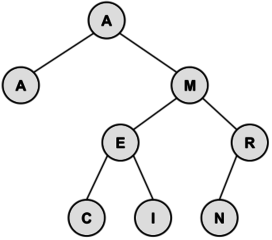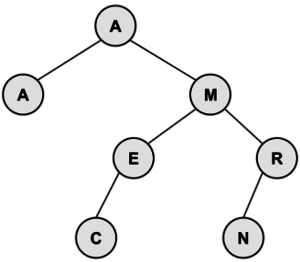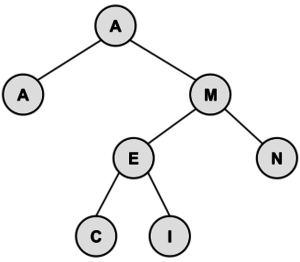## 优先队列

1. 每一个父节点都小于等于它的两个子节点（两个子节点的排放位置不受他们的大小影响）
2. 它的结构是完全二叉树——每一层都完全覆盖，除了叶节点，并且叶节点要从左到右填充

b = bottom-most and right-most element
p = root of tree
p’s key = b’s key
delete b
while (p is larger than either child)
exchange p with smaller child
p = smaller child
end while


## Questions

1. Consider an initially empty stack. After the following operations are performed, what is the value of Z?
PUSH(3)
PUSH(6)
PUSH(8)
Y = POP()
X = POP()
PUSH(X-Y)
Z = POP()

1. Create a min-heap with the letters in the word PROGRAMMING. What are the letters in the bottom-most row, from left to right?

2. Create a binary search tree from the letters in the word PROGRAM. What is the internal path length?

## Solutions

1. The first POP stores 8 in Y. The second POP stores 6 in X. Then, 6-8=-2 is pushed onto the stack. Finally, the last POP removes the -2 and stores it in Z.
2. The bottom row contains the letters RORN, from left-to-right. Here is the entire heap: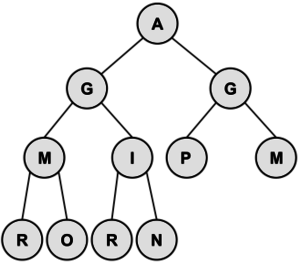3. When drawing the tree, P has a depth of 0, O and R have a depth of 1, G and R have a depth of 2, and A and M have a depth of 3. Therefore, the internal path length is 21 + 22 + 2*3 = 12. Here is the tree: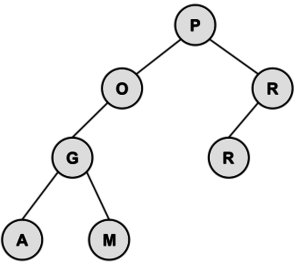# Recursive functions

def Fibonacci(x):
if x == 1 :
return 1
elif x == 2:
return 1
else:
return Fibonacci(x-1) + Fibonacci(x-2)


def Factorial(x):
if x == 0:
return 1
return x * Factorial(x-1)


## Question

1. Find g(11) given the following

$g(x)=\left\{\begin{matrix} g(x-3) + 1 \hspace{1em} if \ x > 0 \\ 3x \hspace{1em} otherwise \end{matrix}\right.$

## Solution

g(11)= g(8)+1
g(8) = g(5)+1
g(5) = g(2)+1
g(2) = g(-1)+1
g(-1)= -3

We now use what we know about g(−1) to learn more values, working our way back "up" the recursion:
We now know the value of g(2):g(2)=−3+1=−2
We now know the value of g(5):g(5)=−2+1=−1
We now know the value of g(8):g(8)=−1+1=0
And finally, we now have the value of g(11):g(11)=0+1=1

For more questions, look at this website.

References

]]>

]]>

NOT GATE: 晶体管有一部分接地，导致没有电流能够通过
AND GATE: 串联，两个晶体管任何一个不通电流就无法通过
OR GATE: 并联，有一边能连通就能连通
XOR: OR GATE与（AND GATE + NOT GATE串联的集合）并联

ALU = 算术逻辑单元

AND, OR, NOT + 判断数字

8 bit ALU

AND电路能一直记录0

Eg：

Multiplexer 多用复路器——将内存地址转换为行和列（就像是在矩阵外面有一个外壳指出每一个bits的位置）

Abstraction

CPU执行——取指令——>解码——>执行（时钟速度1HZ...；超频+降频；动态调整频率）

Bus 总线，链接CPU和内存的线

PCB，不要用电线连接，直接蚀刻到电路板上

1.晶圆上盖氧化层（保护层；可被酸融化）
2.盖光刻胶（被光照射会融化；可以用化学药剂洗掉）
3.盖光掩模（复杂图案，遮住光刻胶，遮住的地方不会融化）
4.用酸溶解氧化层，蚀刻晶圆
5.气体掺杂（改变暴露出来的硅的电学性质）

1、光刻——光的波长相对太长，无法精确雕刻
2、当电子之间隔的距离只有几个原子的时候，电子有可能会发生跃迁，穿过缝隙，导致漏电

1，窗口

2，图标

3，指针

4，菜单

3D图像转2D，计算机一般都通过投影，并且使采用用三角形构图的投影来显示

28 - 30:

IP: 存储发送到的计算机的地址
UDP: 存储端口【标记哪个程序接受】；同时头部还有checksum，即把数据的和加起来与头部这个数据比对，如果相同就表示数据没有损失【只保留16位，超过16舍弃高位】（但是会丢弃损失数据，也不能得知数据包有没有抵达目的地）
TCP: 与UDP相同，但是TCP数据包有编号，意味着接收方能够顺序合成数据包；当通过校验和验证之后会给发送方发送一个接收信号，如果没收到就重发数据；

Cyber-security: 不能向上访问，也不能向下写入

PID - 闭环自动控制技术:
1.计算真实值与理想值比例【如果比例差的大就加大输出】
2.计算积分

3.计算导数 —— 预期控制，如果加速过快（导数过大）就会适当减速

]]>
<![CDATA[ACSL - 4: Assembly Language Programming & LISP]]> https://blog.blinkstar.cn/post/acsl-4 2019-10-11T11:35:24.000Z 汇编语言（英语：assembly language）是一种用于电子计算机、微处理器、微控制器，或其他可编程器件的低级语言。在不同的设备中，汇编语言对应着不同的机器语言指令集。一种汇编语言专用于某种计算机系统结构，而不像许多高级语言，可以在不同系统平台之间移植。
Lisp（历史上拼写为LISP）是具有悠久历史的计算机编程语言家族，有独特和完全括号的前缀符号表示法。起源于公元1958年，是现今第二悠久而仍广泛使用的高端编程语言。

]]>

Lisp（历史上拼写为LISP）是具有悠久历史的计算机编程语言家族，有独特和完全括号的前缀符号表示法。起源于公元1958年，是现今第二悠久而仍广泛使用的高端编程语言。

# 汇编语言

LABEL OPCODE LOC

## OPCODE

STORE 将ACC里的内容放回LOC
SUB ACC里内原有的值会减去LOC的值。结果存储在ACC，LOC保持不变
MULT ACC的值与LOC的值相乘。结果存储在ACC，LOC保持不变
DIV ACC / LOC. 整数部分存储在ACC，LOC不变

.

BG

BE

BL

BU 无条件跳转到BU保存的地址（LABEL）

PRINT

DC

LABEL字段必须要存储常数值，此操作码是必填字段，使用后ACC值不变

END

## Questions

1. 执行完以下程序之后，TEMP的值是什么
 TEMP DC 0 A DC 8 B DC -2 C DC 3 LOAD B MULT C ADD A DIV B SUB A STORE TEMP END
1. 如果以下程序输入值为N，那么X的输出值是什么？用N来表示X
 READ X LOAD X TOP SUB =1 BE DONE STORE A MULT X STORE X LOAD A BU TOP DONE END

## Solutions

1. The ACC takes on values -2, -6, 2, -1, and -9 in that order. The last value, -9, is stored in location TEMP.
2. This program loops between labels TOP and DONE for A times. A has an initial value of X and subsequent terms of N, then values of A-1, A-2, …, 1. Each time through the loop, X is multiplied by the the current value of A. Thus, X = A * (A-1) * (A-2) * … * 1 or X=A! or A factorial. For example, 5! = 5 * 4 * 3 * 2 * 1 = 120. Since the initial value of A is the number input (i.e. N), the algebraic expression is X = N!.

# LISP

## 简介

Lisp（历史上拼写为 LISP）是具有悠久历史的计算机编程语言家族，有独特和完全括号的前缀符号表示法。起源于公元 1958 年，是现今第二悠久而仍广泛使用的高端编程语言。只有 FORTRAN 编程语言比它更早一年。Lisp 编程语族已经演变出许多种方言。现代最著名的通用编程语种是 Clojure、Common Lisp 和 Scheme。

LISP语言的基础就是list。list可以通过把各种元素（数字，文字等）放在括号内，如：

(23 (this is easy) hello 821)

## 函数

### 基本函数

SETQ与SET大致相同，唯一不同之处在于第一个args用SETQ不需要在前面加'

(SET 'a (MULT 2 3)) 6 a = 6
(SET 'a '(MULT 2 3)) (MULT 2 3) a = (MULT 2 3)
(SET 'b 'a) a b = 'a', a character not a mentioned before
(SET 'c a) (MULT 2 3) c = (MULT 2 3)
(SETQ EX (ADD 3 (MULT 2 5))) 13 EX = 13
(SETQ VOWELS '(A E I O U)) (A E I O U) VOWELS = (A E I O U)

(SETQ p '(ADD 1 2 3 4)) (ADD 1 2 3 4) p = (ADD 1 2 3 4)
(ATOM 'p) true ATOM 会判断p是否是ATOM
(ATOM p) NIL 因为p前面没有'，他指的是列表
(EVAL p) 10 EVAL会计算p中表达式的值

### 计算函数

(SUB a b) a - b
(MULT x1 x2 …) 所有参数的积
(DIV a b) a / b
(SQUARE a) a * a
(EXP a n) an
(EQ a b) true如果a和b相等, NIL不相等
(POS a) true如果是正数positive, NIL则是negative
(NEG a) true如果是负数negative, NIL则是positive

(ADD (EXP 2 3) (SUB 4 1) (DIV 54 4)) 24.5
(- (* 3 2) (- 12 (+ 1 2 1))) -2
(ADD (SQUARE 3) (SQUARE 4)) 25

### list function

CAR: 返回list第一个atom
CDR：输出一个list，排除第一个atom
CONS：两个参数，第二个必须是list。CONS会把第一个参数放进第二个列表里的第一个元素
REVERSE：将list的顺序反过来
CADR x = (CAR (CDR x))
CAADDAR x = (CAR (CAR (CDR (CDR (CAR x)))))，以此类推……

(CAR '(This is a list)) This
(CDR '(This is a list)) (is a list)
(CONS 'red '(white blue)) (red white blue)
(SETQ z (CONS '(red white blue) (CDR '(This is a list)))) ((red white blue) is a list)
(REVERSE z) (list a is (red white blue))
(CDDAR z) (blue)

### User-defined Functions

(DEF SECOND (args) (CAR (CDR args)))

## Problems

1. Evaluate the following expression. (MULT (ADD 6 5 0) (MULT 5 1 2 2) (DIV 9 (SUB 2 5)))
2. Evaluate the following expression: (CDR '((2 (3))(4 (5 6) 7)))
3. Consider the following program fragment:
(SETQ X '(RI VA FL CA TX))
(CAR (CDR (REVERSE X)))
What is the value of the CAR expression?

## Solutions

1. (MULT (ADD 6 5 0) (MULT 5 1 2 2) (DIV 6 (SUB 2 5)))
(MULT 11 20 (DIV 6 -3))
(MULT 11 20 -2)
-440

2. The CDR function takes the first element of its parameter (which is assumed to be a list) and returns the list with the first element removed. The first element of the list is (2 (3)) and the list without this element is ((4 (5 6) 7)), a list with one element.
3. The first statement binds variable X to the list '(RI VA FL CA TX). The REVERSE of this list is '(TX CA FL VA RI) whose CDR is '(CA FL VA RI). The CAR of this list is just the atom CA (without the quote).

]]>
<![CDATA[诗与话 - Ⅰ]]> https://blog.blinkstar.cn/post/p-s-1 2019-10-07T13:55:34.000Z 分享一些最近看过的诗和话

]]>

1. 一朵花的美丽在于它曾经凋谢过。

2. 向死而生的意义是：当你无限接近死亡，才能深切体会生的意义。

3. 人活在自己的语言中，语言是人 “存在的家”，人在说话，话在说人。

4. 人安静地生活，哪怕是静静地听着风声，亦能感觉到诗意的生活。

5. 有担当，才有自由。

6. 人生的本质是诗意的，人应该诗意地栖息在大地上。

7. 如果我能向死而生，承认并且直面死亡，我就能摆脱对死亡的焦虑和生活的琐碎。只有这样，我才能自由地做自己。

—— 安东尼奥・拉莫斯・罗萨

—— 老舍

—— 李元胜《如果你试图爱上一个人》

——《你的微笑》聂鲁达

—— 马洛伊・山多尔

—— 迈克尔・坎宁安

—— 丰子恺

—— 亚当・扎加耶夫斯基《铁皮火车》

Late Fragmentby

And did you get what
you wanted from this life, even so?
I did.
And what did you want?
To call myself beloved, to feel myself
beloved on the earth.
—— Raymond Carver

—— 惠特曼

]]>
<![CDATA[我和我的祖国]]> https://blog.blinkstar.cn/post/me-and-my-country 2019-10-06T15:53:39.000Z1. 林治远修理旗杆
2. 高原投身于原子弹事业
3. 冬冬为了给街坊邻居看中国女排世界决赛放弃了去找自己心仪的女孩
4. 香港回归
5. 张北京把自己的北京奥运会票送给了一位四川小孩
6. 两位新疆小伙看见神州十一号返航
7. 2015 年纪念反法西斯战争胜利 70 周年阅兵

3 有一些过分搞笑了，也是深度不太够，感觉有点并走两条线 —— 一是突出中国女排；二是冬冬和小美的故事；但最后效果感觉就是两个内容都没有展现的很完美；

7 的反法西斯战争阅兵觉得没有太看出想从故事表现出主题的什么，虽然是因为主角的保驾护航，阅兵才成功举行，但是感觉展现的内容跟最后的阅兵关联性并不是太大。

]]>

# 给网站加上 Github 贡献表<img src="https://ghchart.rshah.org/you" />

<img src="https://ghchart.rshah.org/EA4C89/you" />

# 给网站添加 fork me on github

<a href="https://github.com/you"><img width="149" height="149" src="https://github.blog/wp-content/uploads/2008/12/forkme_right_white_ffffff.png?resize=149%2C149" class="attachment-full size-full" alt="Fork me on GitHub" data-recalc-dims="1"></a>

# 添加 star,watch,fork,follow

star:

<iframe src="https://ghbtns.com/github-btn.html?user=you&repo=你的仓库&type=star&count=true" frameborder="0" scrolling="0" width="170px" height="20px"></iframe>

watch:

<iframe src="https://ghbtns.com/github-btn.html?user=you&repo=你的仓库&type=watch&count=true&v=2" frameborder="0" scrolling="0" width="170px" height="20px"></iframe>

fork:

<iframe src="https://ghbtns.com/github-btn.html?user=you&repo=你的仓库&type=fork&count=true" frameborder="0" scrolling="0" width="170px" height="20px"></iframe>

follow:

<iframe src="https://ghbtns.com/github-btn.html?user=you&type=follow&count=true" frameborder="0" scrolling="0" width="170px" height="20px"></iframe>

References:

]]>
<![CDATA[读 Educated 有感]]> https://blog.blinkstar.cn/post/educated 2019-09-28T12:53:33.000Z 读完 Educated 很受触动，尤其是后面几章。“What do I need from this place? There was only one thing: my memories.”(Westover 310) 读这段 Tara 写的话，想想自己的过去，我何尝也不是这样呢？过去留下的有什么，只有记忆，也许还有身上的伤疤。有人说 I have changed a lot, becoming more and more outgoing. 也许只有自己知道，自己是那个悄悄在夜晚舔着自己伤口的人。”The distance - physical and mental - that had been traversed in the last decade nearly stopped my breath, and I wondered if perhaps I had changed too much. All my studying, reading, thinking, travelling, had it transformed me into someone who no longer belong anywhere?” (Westover 312)Reference:

1. Educated: A Memoir. New York: Random House, 2018. Westover, Tara. Educated: A Memoir.
]]>
<![CDATA[ACSL - 3: Digital Electronics & Prefix/Infix/Postfix Notation]]> https://blog.blinkstar.cn/post/acsl-3 2019-09-26T11:40:23.000Z

# Digital Electronics

## 定义

BUFFER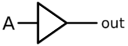X = A
 输出 输出 0 0 1 1
NOT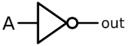X = A
 A X 输出 输出 0 1 1 0
ANDX = AB 或者 A ・ B
 A B X 输出 输出 0 0 0 0 1 0 1 0 0 1 1 1
NAND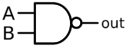X = AB 或者 A ・ B
 A B X 输出 输出 0 0 1 0 1 1 1 0 1 1 1 0
OR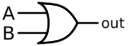X = A+B
 A B X 输出 输出 0 0 0 0 1 1 1 0 1 1 1 1
NORX = A+B
 A B X 输出 输出 0 0 1 0 1 0 1 0 0 1 1 0
XOR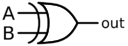X = A ⊕ B
 A B X 输出 输出 0 0 0 0 1 1 1 0 1 1 1 0
XNORX = ~(A ⊕ B) 或 A ⊙ B
 A B X 输出 输出 0 0 1 0 1 0 1 0 0 1 1 1

## 解释

1. 图像后面有点就代表NOT
2. 比较尖的是OR
3. 尖的前面有一条弯的是XOR

# Prefix/Infix/Postfix Notation

## 中缀表达式转前缀表达式（逆波兰式）

1. 初始化两个栈：运算符栈S1和储存中间结果的栈S2；
2. 从右至左扫描中缀表达式；
3. 遇到操作数时，将其压入S2；
4. 遇到运算符时，比较其与S1栈顶运算符的优先级
(4-1)如果S1为空，或栈顶运算符为右括号“)”，则直接将此运算符入栈；
(4-2)否则，若优先级比栈顶运算符的较高或相等，也将运算符压入S1；
(4-3)否则，将S1栈顶的运算符弹出并压入到S2中，再次转到(4-1)与S1
5. 中新的栈顶运算符相比较；
(5)遇到括号时：
(5-1)如果是右括号“)”，则直接压入S1；
(5-2)如果是左括号“(”，则依次弹出S1栈顶的运算符，并压入S2，
(5-3)直到遇到右括号为止，此时将这一对括号丢弃；
6. 重复步骤(2)至(5)，直到表达式的最左边；
7. 将S1中剩余的运算符依次弹出并压入S2；
8. 依次弹出S2中的元素并输出，结果即为中缀表达式对应的前缀表达式。## 中缀表达式转后缀表达式（波兰式）

1. 初始化两个栈：运算符栈 S1 和储存中间结果的栈 S2；
2. 从左至右扫描中缀表达式；
3. 遇到操作数时，将其压入 S2；
4. 遇到运算符时，比较其与 S1 栈顶运算符的优先级：
(4-1) 如果 S1 为空，或栈顶运算符为左括号 “(”，则直接将此运算符入栈；
(4-2) 否则，若优先级比栈顶运算符的高，也将运算符压入 S1（注意转换为前缀表达式时是优先级较高或相同，而这里则不包括相同的情况）；
(4-3) 否则，将 S1 栈顶的运算符弹出并压入到 S2 中，再次转到 (4-1) 与 S1 中新的栈顶运算符相比较；
5. 遇到括号时：
(5-1) 如果是左括号 “(”，则直接压入 S1；
(5-2) 如果是右括号 “)”，则依次弹出 S1 栈顶的运算符，并压入 S2，直到遇到左括号为止，此时将这一对括号丢弃；
6. 重复步骤 (2) 至 (5)，直到表达式的最右边；
7. 将 S1 中剩余的运算符依次弹出并压入 S2；
8. 依次弹出 S2 中的元素并输出，结果的逆序即为中缀表达式对应的后缀表达式（转换为前缀表达式时不用逆序）。## 前缀表达式与后缀表达式辨析

1. 两者符号分别在数字的前面和后面；
2. 中缀表达式转成两者时读取入栈的方向不一样；前缀是倒着读，后缀是正着读；
3. 两者转化时，前缀表达式符号是相同或大于栈顶符号就压入，但后缀表达式一定要大于栈顶符号才压入

# Questions & Solutions

Digital Electronics

References

]]>
<![CDATA[ACSL - 2: Bit-String Flicking & Boolean Algebra]]> https://blog.blinkstar.cn/post/acsl-2 2019-09-25T15:18:05.000Z

# Bit-String Flicking

From helloboyxxx

## Definition

Bit strings (strings of binary digits) are frequently manipulated bit-by-bit using the logical operators not, and, or, and xor. Bits strings are manipulated as a unit using shift and circulate operators

## Bitwise Operators

not (~ or ¬), and (&), or (|), and xor (⊕)

not就是反着来，0变成1，1变成0
e.g. ~100101 = 011010

and就是看两个bit是不是相同的，相同的就是相同的数，不相同就是0
(0 and 0 = 0; 1 and 1 = 0; 1 and 0 = 0; 0 and 1 = 0)
e.g. 1011011 and 011001 = 001001

or就是看两个bit里面有没有1，有就是1，没有就是0
e.g. 1011011 or 011001 = 111011

xor也是看两个bit是不是相同的，相同就是0，不同就是1
e.g. 1011011 xor 011001 = 110010

## Shift Operators

LSHIFT-x and RSHIFT-x

1101101

1101101

LSHIFT-3 x 1101101
1101101
1011010
0110100
1101000

RSHIFT-3 x 11011010
11011010
01101101
00110110
00011011

LCIRC-x 和 RCIRC-x

1101101

1101101
1110110
0111011
1011101

NOT > SHIFT and CIRC > AND > XOR > OR

# Boolean Algebra

xnor = not xor
~x = not x
xor = ⊕
xnor = ⊙

## Laws

 交换律 x + y = y + x x・y = y・x 结合律 (x + y) + z = x + (y + z) x・(y・z) = (x・y)・z 幂等律 x + x = x x・x = x Annihilator Law x + 1 = 1 x・0 = 0 同一律 x + 0 = x x・1 = x 补余律 x + ~x = 1 x・~x = 0 吸收率 x + xy = x x + ~xy = x + y x・(x + y) = x 分配律 x・(y + z) = xy + xz (x + y)・(p + q) = xp + xq + yp + yq (x + y)(x + z)=x + yz 德摩根定律 ~(x + y) = ~x・~y ~(x・y} = ~x + ~y 双重否定律 ~~x = x XOR 和 XNOR 关系 x ⊙ y = ~(x ⊕ y)= x ⊕ ~y =~x ⊕ y

## 解释

1. 交换律——类似于加减乘除
2. 结合律——类似于加减乘除
3. 幂等律——x and x = x; x or x = x
4. Annihilator Law——x or 1 = 1; x and 0 = 0
5. 同一律——x or 0 = 0; x and 1 = x
6. 补余律——x or not x = 1(if x = 0, not x = 1; if x = 1, not x = 0); x and not x = 0
7. 吸收率——x + xy = x(1 + y) = 1(because 1 + y ≥ 1); (x + y)(x + z) = x・x + x・z + x・y + y・z = x + y・z(x(1 + y + z) = 1)
8. 德摩根定律——可以通过列表证明(不严谨);
严谨证明：9. not not x = x
10. by definition, xnor is not xor;

# Problems

1. Evaluate the following expression:
(0101110 AND NOT 110110 OR (LSHIFT-3 101010))
2. Evaluate the following expression:
(RSHIFT-1 (LCIRC-4 (RCIRC-2 01101)))
3. List all possible values of x (5 bits long) that solve the following equation.
(LSHIFT-1 (10110 XOR (RCIRC-3 x) AND 11011)) = 01100
4. Evaluate the following expression:
((RCIRC-14 (LCIRC-23 01101)) | (LSHIFT-1 10011) & (RSHIFT-2 10111))
5. Simplify the following expression as much as possible:6.# Solutions

1. The expression evaluates as follows:
(0101110 AND 001001 OR (LSHIFT-3 101010))
(001000 OR (LSHIFT-3 101010))
(001000 OR 010000)
011000
2. The expression evaluates as follows, starting at the innermost parentheses:
(RCIRC-2 01101) => 01011
(LCIRC-4 01011) => 10101
(RSHIFT-1 10101) = 01010
3. Since x is a string 5 bits long, represent it by abcde. (RCIRC-3 x) is cdeab which, when ANDed with 11011 gives cd0ab. This is XORed to 10110 to yield Cd1Ab (the capital letter is the NOT of its lower case). Now, (LSHIFT-1 Cd1Ab) = d1Ab0 which has a value of 01100, we must have d=0, A=1 (hence a=0), b=0. Thus, the solution must be in the form 000, where * is an “I-don’t-care”. The four possible values of x are: 00000, 00001, 00100 and 00101.
4. The problem can be rewritten as

A | B & C
The AND has higher precedence than the OR.

The evaluation of expression A can be done in a straightforward way: (LCIRC-23 01101) is the same as (LCIRC-3 01101) which has a value of 01011, and (RCIRC-14 01011) is the same as (RCIRC-4 01011) which has a value of 10110. Another strategy is to offset the left and right circulates. So, ((RCIRC-14 (LCIRC-23 01101)) has the same value as (LCIRC-9 01101), which has the same value as (LCIRC-4 01101) which is also 11010.

Expressions B and C are pretty easy to evaluate:

B = (LSHIFT-1 10011) = 00110
C = (RSHIFT-2 10111) = 00101
The expression becomes

A | B & C = 10110 | 00110 & 00101 = 10110 | 00100 = 10110
5.6.References

]]>

]]>

]]>
<![CDATA[ACSL - 1: Computer Number System & Graph Theory]]> https://blog.blinkstar.cn/post/acsl-1 2019-09-23T14:45:50.000Z

# Computer Number System

From helloboyxxx

## 二进制

1 1 0 1# Graph Theory

## Overview

V = {v1,v2,v3,...vn,} 是由 G 的结点 (vertex or node) 组成的集合
E = {e1,e2,e3,...en} 是由连接两个结点的边 (edge) 组成的集合

## 树## 有向图V={A,B,C,D,E}
E={AB,AC,AE,CD,DE,ED,DB}

## 邻接矩阵$\begin{bmatrix} 0 & 1 & 1 & 0 & 1\\ 0& 0 & 0 & 0 & 0\\ 0 & 0 & 0 & 1 & 0\\ 0 & 1 & 0 & 0 & 1\\ 0 & 0 & 0 & 1 & 0 \end{bmatrix}$

## 遍历性A有3条边连接
B有3条边连接
C有5条边连接

# Problems

1. Solve for x where x16=36768.

3. Find the number of different cycles contained in the directed graph with vertices {A, B, C, D, E} and edges {AB, BA, BC, CD, DC, DB, DE}.

4. In the following directed graph, find the total number of different paths from vertex A to vertex C of length 2 or 4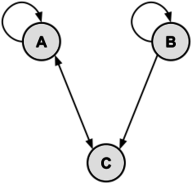5. Given the adjacency matrix, draw the directed graph.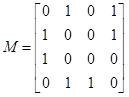# Solutions

1. One method of solution is to convert 36768 into base 10, and then convert that number into base 16 to yield the value of x.
An easier solution, less prone to arithmetic mistakes, is to convert from octal (base 8)to hexadecimal (base 16) through the binary (base 2) representation of the number:

\begin{aligned} 3676_8 &= 011\; 110\; 111\; 110_2\\ &=0111\; 1011\; 1110_2\\ &=7BE_{16} \end{aligned}

1. One could convert the hex numbers into base 10, perform the subtraction, and then convert the answer back to base 16. However, working directly in base 16 isn't too hard. As in conventional decimal arithmetic, one works from right-to-left, from the least significant digits to the most.

The rightmost digit becomes 2, because D-B=2.
The next column is A-E. We need to borrow a one from the 5 column, and 1A-E=C
In the next column, 4-9=B, again, borrowing a 1 from the next column.
Finally, the leftmost column, E-6=8

Combining these results of each column, we get a final answer of 8BC216.

2. The graph is as follows: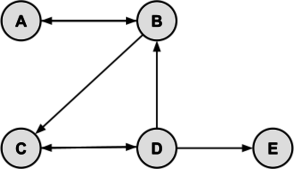By inspection, the cycles are: ABA, BCDB, and CDC. Thus, there are 3 cycles in the graph.

3. Let matrix M represent the graph. Recall that the number of paths from vertex i to vertex j of length p equals Mp. The values of M, M2 and M4 are: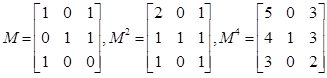There is 1 path of length 2 from A to C (cell [1,3]) and 3 paths of length 4 (cell [1,3]).

4. There must be exactly 4 vertices: V = {A, B, C, D}. There must be be exactly 7 edges: E = {AB, AD, BA, BD, CA, DB, DC}. Here are two valid drawings of the graph: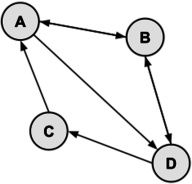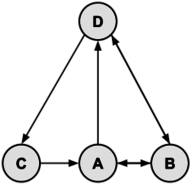References

]]>
<![CDATA[ACSL 目录]]> https://blog.blinkstar.cn/post/acsl-intro 2019-09-23T14:39:47.000Z ACSL organizes computer science contests and computer programming contests for elementary, junior, and senior high school students.

]]>
ACSL organizes computer science contests and computer programming contests for elementary, junior, and senior high school students.

]]>

2、《青春颂歌》

3、学校
—— 金子美铃

䴙䴘和青蛙都在叫

4、等候（节选）

5、Gibran《Sand and foam》
Give me silence, I will challenge the night

6、Walden Pond
by Henry David Thoreau
I went to the woods because I wished to live deliberately, to front only the essential facts of life, and see if I could not learn what it had to teach, and not, when I came to die, discover that I had not lived.

By Robert Frost
Two roads diverged in a wood, and I took the one less traveled by, And that has make all the difference.

8、Infinitive
By Giacomo Leopardi
And as the wind
Comes through the copses sighing to my ears,
The infinite silence and the passing voice
I must compare: remembering the seasons,
Quiet in dead eternity, and the present,
Living and sounding still. And into this
Immensity my thought sinks ever drowning,
And it is sweet to shipwreck in such a sea.

9、在这一刻 - 海桑

]]>

O Captain! My Captain!

]]>

Gridea-theme bitcron-pro

1、图片灯箱
2、底部添加 “重拾写作的乐趣”
3、点击爱心特效
4、网站运行时间计算（如果要看怎么改运行时间可以参考这篇文章 ）
5、阅读时间以及文章字数计算
6、toc 目录支持 —— 在 Gridea 内 @[toc] 即可
7、头像旋转特效
8、暗黑模式
9、添加更多的社交样式
10、网站总访问量统计
11、版权说明
12、繁简体转换
13、字体缩小
14、Katex 支持（详情可以查看这篇文章）
15、增加显示封面的功能 [注：如果没有设置封面图默认会使用 unsplash 图片]
16、搜索功能（感谢云中君；详情可参考这篇文章 ; 可在设置里选择关闭)
17、文章内上下篇显示
18、微信、百度等防盗链解除（注：在微信 X5 内核浏览器等一些浏览器无效；实测 Chrome 下正常）
19、代码高亮
20、添加图片简介
21、无法加载的图片自动替换成 404
22、发布时间过长的文章增加提示
23、文章目录贴边显示
24、特殊功能支持 —— 圣诞特效

2019.12.25
1、添加圣诞特效

2019.12.13
1、文章右侧添加文章目录

2019.12.8
1、增加图片灯箱效果
2、删除 Pjax 效果，因为对一些 js 不兼容

2019.11.28
1、修复 pjax bug
2、在主页文章添加文章字数显示
3、更换文章页面字数显示的小图标
4、修复赞赏码显示问题

English_version
Based on original version add these changes:
4、Count website run time
5、Count reading time and word number
9、Add more social media icon at footer
13、Add support for decreasing font size
19、Highlight code
21、Replace broken picture with 404 picture
22、Add prompt for article released for a long time
24、Special feature - Christmas features

Update
2019.12.25
1、Special feature - Christmas features

2019.12.13

2019.12.8
1、Add support for picture light box
2、Cancel Pjax function because it doesn't support some js

2019.11.28
1、Fix pjax bug
4、Fix display of donate qrcode

*诗 - 赞赏 1 元

]]>

……

……

]]>

]]>

—— 惠特曼

（我热烈地爱过某个人，我的爱情没有受到青睐，

（我仍然肩负着我多年喜爱的责任，

“从此刻起我规定自己摆脱羁绊和虚构的限制，

—— 节选自《大路之歌》

By Walt Whitman
AFOOT and light-hearted, I take to the open road,
Healthy, free, the world before me,
The long brown path before me, leading wherever I choose.
Henceforth I ask not good-fortune—I myself am good fortune;
Henceforth I whimper no more, postpone no more, need nothing,
Strong and content, I travel the open road.
The earth—that is sufficient;
I do not want the constellations any nearer;
I know they are very well where they are;
I know they suffice for those who belong to them.
(Still here I carry my old delicious burdens,
I carry them, men and women,
I carry them with me wherever I go,
I swear it is impossible for me to get rid of them,
I am fill’d with them,
and I will fill them in return.)

From this hour, freedom!
From this hour I ordain myself loos’d of limits and imaginary lines,
Going where I list, my own master, total and absolute,
Listening to others, and considering well what they say,
Pausing, searching, receiving, contemplating,
Gently, but with undeniable will, divesting myself of the holds that would hold me.

“我想在大路上不管遇见什么，我都会喜欢，遇见我的人也都会喜欢我，我想我看见的人必定幸福。” 这描述的也是一种乐观自由的心态，也并不是真正的在大路上看到的每一个人都很幸福。但因为我们是幸福的、是自由的，所以看任何东西都是快乐的。这也正是我们在心路上要追求的。

]]>

]]>

# 正文

## 1. WPD

WPD is the most convenient and proper way to customize privacy related settings in Windows.## 2. DWS

Destroy Windows 10 Spying，真正的win10间谍杀手1、win+R（打开运行）
2、输入services.msc
3、找到Windows Biometric Service，重启该服务即可

1903包括以上使用了DWS就会导致无法使用人脸/指纹解锁（重启服务也没办法），但总是有办法解决的，下面这个软件就可以解决这个问题——

## 3. O&O ShutUp10## 4. win10 隐私保护及病毒和威胁防护工具# 结语

]]>

]]>]]>
<![CDATA[Pjax]]> https://blog.blinkstar.cn/post/pjax 2019-08-17T03:50:47.000Z 近几天为了加快博客访问速度想尽了办法，首先是用了 InstantClick 但是引用之后点击链接总是会显示空白页，Console 里面提示 Cannot read property 'appendChild' of null。最后去翻了 github 上的 issue 也没有找到解决办法，只能放弃。有兴趣的可以戳这里了解这个项目。

<script type="text/javascript" src="jquery.js地址" </script> //必须要调用jquery
<script type="text/javascript" src="jquery.pjax.min.js地址"></script>
<script>
$(document).pjax('a[target!=_blank]', '#pjax-container', { fragment: '#pjax-container' }); </script>  1. 因为我的博客用了 katex 和 busuanzi 的统计，所以需要在 pjax 的回调里面回调这两个 js 文件，加上以下的代码 <script type="text/javascript">$(document).on('pjax:complete', function(){
pjax();
});
$(window).on('popstate.pjax', function () { pjax(); }) function pjax(){$.getScript("https://busuanzi.ibruce.info/busuanzi/2.3/busuanzi.pure.mini.js");
$.getScript("https://cdn.bootcss.com/KaTeX/0.10.2/contrib/auto-render.min.js"); } </script>  完整的代码如下： <script type="text/javascript">$(document).pjax('a[target!=_blank]', '#pjax-container', {
fragment: '#pjax-container'
});
$(document).on('pjax:complete', function(){ pjax(); });$(window).on('popstate.pjax', function () {
pjax();
})
function pjax(){
$.getScript("https://busuanzi.ibruce.info/busuanzi/2.3/busuanzi.pure.mini.js");$.getScript("https://cdn.bootcss.com/KaTeX/0.10.2/contrib/auto-render.min.js");
}
</script>

]]>
<![CDATA[Katex]]> https://blog.blinkstar.cn/post/katex 2019-08-16T13:15:56.000Z KaTeX – The fastest math typesetting library for the web.

]]>
KaTeX – The fastest math typesetting library for the web.

<link rel="stylesheet" href="https://cdn.jsdelivr.net/npm/katex@0.11.1/dist/katex.min.css" integrity="sha384-zB1R0rpPzHqg7Kpt0Aljp8JPLqbXI3bhnPWROx27a9N0Ll6ZP/+DiW/UqRcLbRjq" crossorigin="anonymous">
<script defer src="https://cdn.jsdelivr.net/npm/katex@0.11.1/dist/katex.min.js" integrity="sha384-y23I5Q6l+B6vatafAwxRu/0oK/79VlbSz7Q9aiSZUvyWYIYsd+qj+o24G5ZU2zJz" crossorigin="anonymous"></script>
<script defer src="https://cdn.jsdelivr.net/npm/katex@0.11.1/dist/contrib/auto-render.min.js" integrity="sha384-kWPLUVMOks5AQFrykwIup5lo0m3iMkkHrD0uJ4H5cjeGihAutqP0yW0J6dpFiVkI" crossorigin="anonymous" onload="renderMathInElement(document.body);"></script>


<link href="https://cdn.bootcss.com/KaTeX/0.11.1/katex.min.css" rel="stylesheet">
<script src="https://cdn.bootcss.com/KaTeX/0.11.1/katex.min.js"></script>
<script src="https://cdn.bootcss.com/KaTeX/0.11.1/contrib/auto-render.min.js"></script>


$J(\theta) = J(\theta_0, \theta_1) = \frac{1}{2m}\sum_{i=1}^m(h(x^{(i)}) - y^{(i)})^2$

Sigmoid函数：$g (z) = \frac {1}{1 + e^{-z}}$
SVM成本函数：

$J(\theta) = C \left[ \sum_{i=1}^m y^{(i)} cost_1(\theta^T x^{(i)}) + (1 - y^{(i)}) cost_0(\theta^T x^{(i)}) \right] + \frac{1}{2} \sum_{j=1}^n \theta_j^2$

K-mean成本函数：$J = \frac{1}{m} \sum_{i=1}^m \| x^{(i)} - u_{c^{(i)}} \|^2$

]]>

]]>
“桂林山水甲天下”

## 有幸趁现在来了桂林 —— 题记

]]>

]]>

1、鼠标点击爱心

!function(e, t, a) {    function r() {        for (var e = 0; e < s.length; e++) s[e].alpha <= 0 ? (t.body.removeChild(s[e].el), s.splice(e, 1)) : (s[e].y--, s[e].scale += .004, s[e].alpha -= .013, s[e].el.style.cssText = "left:" + s[e].x + "px;top:" + s[e].y + "px;opacity:" + s[e].alpha + ";transform:scale(" + s[e].scale + "," + s[e].scale + ") rotate(45deg);background:" + s[e].color + ";z-index:99999");        requestAnimationFrame(r)    }    function n() {        var t = "function" == typeof e.onclick && e.onclick;        e.onclick = function(e) {            t && t(),            o(e)        }    }    function o(e) {        var a = t.createElement("div");        a.className = "heart",        s.push({            el: a,            x: e.clientX - 5,            y: e.clientY - 5,            scale: 1,            alpha: 1,            color: c()        }),        t.body.appendChild(a)    }    function i(e) {        var a = t.createElement("style");        a.type = "text/css";        try {            a.appendChild(t.createTextNode(e))        } catch(t) {            a.styleSheet.cssText = e        }        t.getElementsByTagName("head").appendChild(a)    }    function c() {        return "rgb(" + ~~ (255 * Math.random()) + "," + ~~ (255 * Math.random()) + "," + ~~ (255 * Math.random()) + ")"    }    var s = [];    e.requestAnimationFrame = e.requestAnimationFrame || e.webkitRequestAnimationFrame || e.mozRequestAnimationFrame || e.oRequestAnimationFrame || e.msRequestAnimationFrame ||    function(e) {        setTimeout(e, 1e3 / 60)    },    i(".heart{width: 10px;height: 10px;position: fixed;background: #f00;transform: rotate(45deg);-webkit-transform: rotate(45deg);-moz-transform: rotate(45deg);}.heart:after,.heart:before{content: '';width: inherit;height: inherit;background: inherit;border-radius: 50%;-webkit-border-radius: 50%;-moz-border-radius: 50%;position: fixed;}.heart:after{top: -5px;}.heart:before{left: -5px;}"),    n(),    r()} (window, document);

<script type="text/javascript"> src=js文件地址></script>

2、本站运行时间

本站已稳定运行了<script type="text/javascript"><strong>var urodz= new Date("10/29/2018"); //网站建站时间，月/日/年var now = new Date();var ile = now.getTime() - urodz.getTime();var dni = Math.floor(ile / (1000 * 60 * 60 * 24));document.write(+dni)</strong></script>天

3、输入框输入特效

<script src=js文件地址</script><script>POWERMODE.colorful = true; POWERMODE.shake = false;document.body.addEventListener('input', POWERMODE);</script>

4、阅读时间计算

<script>var readTime = document.getElementById("nfont").innerText.length; //把文章主体内容id设置为nfontvar readTime = readTime / 250;var readTime = Math.round(readTime);if(readTime > 1){    document.getElementById("read_time").innerHTML = '阅读时长 ' + readTime + "分钟" //把需要显示时间的地方id设置为read_time}else{    document.getElementById("read_time").innerHTML = '阅读时长 1分钟'}</script>

var data=document.getElementById("nfont").innerTextvar pattern = /[a-zA-Z0-9_\u0392-\u03c9]+|[\u4E00-\u9FFF\u3400-\u4dbf\uf900-\ufaff\u3040-\u309f\uac00-\ud7af]+/g;var m =data.match(pattern);var count = 0;if(m==null){document.getElementById("fn").innerHTML = count + "字"}else{for(var i=0;i<m.length;i++){    if(m[i].charCodeAt(0)>=0x4E00){        count +=m[i].length;    }else{        count += 1;    }}var readTime = count / 250;var readTime = Math.round(readTime);if(readTime > 1){    document.getElementById("read_time").innerHTML = '阅读时长 ' + readTime + "分钟" //把需要显示时间的地方id设置为read_time}else{    document.getElementById("read_time").innerHTML = '阅读时长 1分钟'}}

5、头像点击旋转 90°(Reference)

<img id="img1" style="display:inline-block;" src="头像地址"/> //<script>var r = 0;    window.onload = function(){        var current = 0;        document.getElementById('img1').onclick = function(){            current = (current+90)%360;            this.style.transform = 'rotate('+current+'deg)';        }    };</script>

6、在博客底部加入 “重拾写作的乐趣”

<img src="svg地址">

7、加入暗黑模式

<script type="text/javascript" src="js地址"></script><script>var options = {  bottom: '64px', // default: '32px'  right: '32px', // default: '32px'  left: 'unset', // default: 'unset'  time: '0.5s', // default: '0.3s'  mixColor: '#fff', // default: '#fff'  backgroundColor: '#fff',  // default: '#fff'  buttonColorDark: '#100f2c',  // default: '#100f2c'  buttonColorLight: '#fff', // default: '#fff'  saveInCookies: false, // default: true,  label: '🌓', // default: ''  autoMatchOsTheme: true // default: true}const darkmode = new Darkmode(options);darkmode.showWidget();</script>

]]>
<![CDATA[机器学习 - SVM-1]]> https://blog.blinkstar.cn/post/ji-qi-xue-xi-svm-1 2019-08-12T02:53:05.000Z 支持向量机（Support Vector Machine, SVM）是一类按监督学习（supervised learning）方式对数据进行二元分类（binary classification）的广义线性分类器（generalized linear classifier），其决策边界是对学习样本求解的最大边距超平面（maximum-margin hyperplane）。

]]>

# 正文

## 概念

$d=\left| \frac{Ax_{0}^{}+By_{0}^{}+C}{\sqrt{A_{}^{2}+B_{}^{2}}} \right|$

$d=\frac{w_{}^{T}A+b}{\lVert w \rVert}$

$d=\frac{1}{\lVert w \rVert}$

$y_{}^{\left( i \right)}\left( w_{}^{T}x_{}^{\left( i \right)}+b \right) \ge 1$

$y_{}^{\left( i \right)}\left( w_{}^{T}x_{}^{\left( i \right)}+b \right) \ge 1$

## 线性不可分

$arg\min\text{\ }\lVert w_{}^{2} \rVert +R\sum_{i=1}^m{\varepsilon _{i}^{}}$

$y_{}^{\left( i \right)}\left( w_{}^{T}x_{}^{\left( i \right)}+b \right) \ge 1-\varepsilon$

$y_{}^{\left( i \right)}\left( w_{}^{T}x_{}^{\left( i \right)}+b \right)$

$y_{}^{\left( i \right)}\left( w_{}^{T}x_{}^{\left( i \right)}+b \right) \ge 1$

]]>
<![CDATA[Night at HongKong]]> https://blog.blinkstar.cn/post/night-at-hongkong 2019-08-09T13:19:57.000Z Miss

HongKong's night
Hotel's light
Silent tonight
Wait for you two fortnight

Walk through the arch
Strange marks on dark
Some dogs bark
Two hearts spark
Try to be a Stark

Then
never mind
never back
never miss

]]>
Back to dream

Wind passes by
Man goes away
Never back...


One day we will Back To Our Dream

]]>

]]>1. 显摆——发一些技术含量高+看起来很牛的朋友圈

2. 孤独

3. 传播

4. 记录生活]]>
<![CDATA[飞鸟集]]> https://blog.blinkstar.cn/post/stray-bird 2019-08-02T04:41:20.000Z In memory of Stray Bird, in memory of Rabindranath Tagore

]]>
In memory of Stray Bird, in memory of Rabindranath Tagore

If you shed tears when you miss the sun, you also miss the stars.

Te fsh in the water is silent, the animal on the earth is noisy, the bird in the air is singing.
But Man has in him the silence of the sea, the noise of the earth and the music of the air.

Like the meeting of the seagulls and the waves we meet and come near.
Te seagulls fy of, the waves roll away and we depart.

Let life be beautiful like summer fowers and death like autumn leaves.

I have my stars in the sky.
but oh for my little lamp unlit in my house.

Te sunshine greets me with a smile.
Te rain, his sad sister, talks to my heart.

"I have lost my dewdrop," cries the fower to the morning sky that has lost all its stars.

Tis life is the crossing of a sea, where we meet in the same narrow ship.
In death we reach the shore and go to our diferent worlds.

I live in this little world of mine and am afraid to make it the least less.
Lift me into thy world and let me have the freedom gladly to lose my all.

One word keep for me in thy silence, O World, when I am dead, "I have loved."

Let the dead have the immortality of fame, but the living the immortality of love.

I shall die again and again to know that life is inexhaustible.

Tat love can ever lose is a fact that we cannot accept as truth.

I long for the Island of Songs across this heaving sea of shouts.

I have sufered and despaired and known death and I am glad that I am in this great world.

End---

Let this be my last word, that I trust thy love.

]]>
<![CDATA[机器学习 - 决策树]]> https://blog.blinkstar.cn/post/ji-qi-xue-xi-dtree 2019-07-29T03:30:17.000Z 决策树是一种常见的机器学习算法，它的思想十分朴素，类似于我们平时利用选择做决策的过程。

]]>

# 正文

## 信息熵

1948 年，香农提出了信息熵的概念解决了信息的量化问题【我们今天知道的1bit就是信息量化的结果】（如果不知道什么是熵的读者可以戳这里）。信息熵是被用来衡量信息的不确定性的东西，根据常识我们也可以知道，当一个东西不确定性越大的时候，我们想要了解它就要知道更多的信息。信息熵根据定义为如下表达式：

$H\left( X \right) =-\sum_{x\in X}^{}{P\left( x \right) \log _{2}^{}P\left( x \right)}$

$H\left( X \right) =-\left( \frac{1}{2}\log _{2}^{}\frac{1}{2}+\frac{1}{2}\log _{2}^{}\frac{1}{2} \right) =1$

$H\left( D_{base}^{} \right) =-\left( \frac{2}{16}\log _{2}^{}\frac{2}{16}+\frac{2}{16}\log _{2}^{}\frac{2}{16}+\frac{4}{16}\log _{2}^{}\frac{4}{16}+\frac{8}{16}\log _{2}^{}\frac{8}{16} \right) =1.75$

$H\left( D1_{sub1}^{} \right) =-\left( \frac{2}{6}\log _{2}^{}\frac{2}{6}+\frac{4}{6}\log _{2}^{}\frac{4}{6} \right) =0.918296$

$H\left( D1_{sub2}^{} \right) =-\left( \frac{2}{10}\log _{2}^{}\frac{2}{10}+\frac{8}{10}\log _{2}^{}\frac{8}{10} \right) =0.721928$

## 决策树实现

### 3、基尼不纯度

$Gini\left( D \right) =\sum_{x\in X}^{}{P\left( x \right) \left( 1-P\left( x \right) \right) =1-\sum_{x\in X}^{}{P\left( x \right) _{}^{2}}}$

### 4、剪枝算法

]]>
<![CDATA[机器学习 - 逻辑回归]]> https://blog.blinkstar.cn/post/ji-qi-xue-xi-logistic 2019-07-27T07:51:13.000Z Logistic 回归又称 logistic 回归分析，是一种广义的线性回归分析模型，常用于数据挖掘，疾病自动诊断，经济预测等领域。

]]>
Logistic 回归又称 logistic 回归分析，是一种广义的线性回归分析模型，常用于数据挖掘，疾病自动诊断，经济预测等领域。

# 正文

## 预测函数——二元分类

$g\left( z \right) =\frac{1}{1+e_{}^{-z}}$

$h_{\theta}^{}\left( x \right) =\theta _{}^{T}x$

$h_{\theta}^{}\left( x \right) =g\left( z \right) =g\left( \theta _{}^{T}x \right) =\frac{1}{1+e_{}^{-\theta _{}^{T}x}}$

$g\left( -\theta _{1}^{}x+\theta _{2}^{} \right) =\frac{1}{1+e_{}^{-\left( \theta _{1}^{}x+\theta _{2}^{} \right)}}$

h(x)表示在输入值为x，参数为θ的前提条件下y=1的概率。用概率论的公式可以写成

$h_{\theta}^{}\left( x \right) =P\left( y=1|x,\theta \right)$

## 边界

$h_{\theta}^{}\left( x \right) =g\left( z \right) =g\left( \theta _{}^{T}x \right) =\frac{1}{1+e_{}^{-\theta _{}^{T}x}}$

## 成本函数

$\cos t\left( h_{\theta}^{}\left( x \right) ,y \right) =\begin{cases} -\log \left( h_{\theta}^{}\left( x \right) \right)& if\ y=1\\ -\log \left( 1-h_{\theta}^{}\left( x \right) \right)& if\ y=0\\ \end{cases}$

$\cos t\left( h_{\theta}^{}\left( x \right) ,y \right) =-y\log \left( h_{\theta}^{}\left( x \right) \right) -\left( 1-y \right) \log \left( h_{\theta}^{}\left( x \right) \right)$

$J\left( \theta \right) =-\frac{1}{m}\left( \sum_{i=1}^m{y_{i}^{}\log \left( h_{\theta}^{}\left( x_{i}^{} \right) \right) +\left( 1-y_{i}^{} \right) \log \left( 1-h_{\theta}^{}\left( x_{i}^{} \right) \right)} \right)$

## 梯度下降算法

$g\left( -\theta _{1}^{}x+\theta _{2}^{} \right) =\frac{1}{1+e_{}^{-\left( \theta _{1}^{}x+\theta _{2}^{} \right)}}$

$\theta _1=\theta _1-\alpha \frac{\partial}{\partial \theta _{1}^{}}J\left( \theta \right)$

$\theta _0=\theta _0-\alpha \frac{\partial}{\partial \theta _{0}^{}}J\left( \theta \right)$

$\theta _1=\theta _1-\alpha \frac{1}{m}\sum_{i=1}^m{\left( h_{\theta}\left( x^{\left( i \right)} \right) -y^{\left( i \right)} \right)}x_{}^{\left( i \right)}$

$\theta _0=\theta _0-\alpha \frac{1}{m}\sum_{i=1}^m{\left( h_{\theta}\left( x^{\left( i \right)} \right) -y^{\left( i \right)} \right)}$

\begin{aligned} \sigma \left( x \right) ’&=\left( \frac{1}{1+e^{-x}} \right) ’=\frac{-\left( 1+e^{-x} \right) ’}{\left( 1+e^{-x} \right) ^2}=\frac{-1’-\left( e^{-x} \right) ’}{\left( 1+e^{-x} \right) ^2}\\ &=\frac{0-\left( -x \right) ’\left( e^{-x} \right)}{\left( 1+e^{-x} \right) ^2}=\frac{-\left( -1 \right) \left( e^{-x} \right)}{\left( 1+e^{-x} \right) ^2}=\frac{e^{-x}}{\left( 1+e^{-x} \right) ^2}\\ &=\left( \frac{1}{1+e^{-x}} \right) \left( \frac{e^{-x}}{1+e^{-x}} \right) =\sigma \left( x \right) \left( \frac{+1-1+e^{-x}}{1+e^{-x}} \right)\\ &=\sigma \left( x \right) \left( \frac{1+e^{-x}}{1+e^{-x}}-\frac{1}{1+e^{-x}} \right)\\ &=\sigma \left( x \right) \left( 1-\sigma \left( x \right) \right)\\ \end{aligned}

## 正则化

### 线性回归正则化

$J\left( \theta \right) =\frac{1}{2m}\left[ \sum_{i=1}^m{\left( h_{\theta}^{}\left( x_{i}^{} \right) -y_{i}^{} \right) _{}^{2}} \right] +\lambda \sum_{j=1}^n{\theta _{j}^{2}}$

$\theta _{j}^{}=\theta _{j}^{}-a\frac{1}{m}\sum_{i=1}^m{\left[ \left( \left( h\left( x_{}^{\left( i \right)} \right) -y_{}^{\left( i \right)} \right) x_{j}^{\left( i \right)} \right) +\frac{\lambda}{m}\theta _{j}^{} \right]}$

### 逻辑回归正则化

$J\left( \theta \right) =-\frac{1}{m}\left[ \sum_{i=1}^m{y_{}^{\left( i \right)}\log \left( h_{\theta}^{}\left( x_{}^{\left( i \right)} \right) \right) +\left( 1-y_{}^{\left( i \right)} \right) \log \left( 1-h_{\theta}^{}\left( x_{}^{\left( i \right)} \right) \right)} \right] +\frac{\lambda}{2m}\sum_{j=1}^n{\theta _{j}^{2}}$

$\theta _{j}^{}=\theta _{j}^{}\left( 1-\alpha \frac{\lambda}{m} \right) -\alpha \frac{1}{m}\sum_{i=1}^m{\left( \left( h\left( x_{}^{\left( i \right)} \right) -y_{}^{\left( i \right)} \right) x_{j}^{\left( i \right)} \right)}$

## 范数

L1范数（L1正则项 ：向量里元素的绝对值之和
||θ||1 = ||θ1||+||θ2||
L2范数（L2正则项 ：向量里元素的平方和的开方根
||θ||2 = √θ12 + θ22
L1 范数作为正则项，会让模型参数稀疏化，即让模型参数向量里为0的元素尽量多。而L2范数作为正则项，会让模型参数尽量小，但不会为0，即尽量让每个特征对预测值都有贡献。

]]>
<![CDATA[机器学习 - 线性回归]]> https://blog.blinkstar.cn/post/ji-qi-xue-xi-linear 2019-07-26T02:53:17.000Z 线性回归是利用数理统计中回归分析，来确定两种或两种以上变量间相互依赖的定量关系的一种统计分析方法，运用十分广泛。

]]>

# 前言

## 微积分基础知识

$\frac{\partial}{\partial x}f\left( x \right) =cnx_{}^{n-1}$

$\frac{\partial}{\partial x_{0}^{}}\sum_{i=0}^n{F\left( x_{i}^{} \right) =\sum_{i=0}^n{\frac{\partial}{\partial x_{0}^{}}F\left( x_{i}^{} \right)}}$

$\frac{\partial}{\partial x}J\left( x \right) =g\left( f\left( x \right) \right) '\times f\left( x \right) '$

$\frac{\partial}{\partial x}f\left( x,y \right) =nax_{}^{n-1}$

$\frac{\partial}{\partial y}f\left( x,y \right) =mby_{}^{m-1}$

# 正文

## 成本函数

$J\left( \theta \right) =\frac{1}{2m}\sum_{i=1}^m{\left( h_{\theta}^{}\left( x_{i}^{} \right) -y_{i}^{} \right) _{}^{2}}$

## 梯度下降算法

$\theta _{j}^{}=\theta _{i}^{}-\alpha \times Gradient$

$Gradient=\frac{\partial}{\partial \theta _{j}^{}}J\left( \theta \right)$

$\theta _j=\theta _j-\alpha \frac{\partial}{\partial \theta _j}J\left( \theta \right)$

\begin{aligned} \theta _0&=\theta _0-\frac{\alpha}{m}\sum_{i=1}^m{\left( h\left( x_{}^{i} \right) -y_{}^{i} \right)}\\ \end{aligned}

\begin{aligned} \theta _j&=\theta _j-\frac{\alpha}{m}\sum_{i=1}^m{\left( \left( h\left( x^{\left( i \right)} \right) -y^{\left( i \right)} \right) x_{j}^{\left( i \right)} \right)}\\ \end{aligned}

\begin{aligned} \frac{\partial}{\partial \theta _j}J\left( \theta \right) &=\frac{\partial}{\partial \theta _j}\frac{1}{2m}\sum_{i=1}^m{\left( h\left( x^{\left( i \right)} \right) -y^{\left( i \right)} \right)}^2\\ &=\frac{1}{2m}\sum_{i=1}^m{\frac{\partial}{\partial \theta _j}}\left( h\left( x^{\left( i \right)} \right) -y^{\left( i \right)} \right) ^2\\ &=2\frac{1}{2m}\sum_{i=1}^m{\left( \left( h\left( x^{\left( i \right)} \right) -y^{\left( i \right)} \right) \frac{\partial}{\partial \theta _j}\left( h\left( x^{\left( i \right)} \right) -y^{\left( i \right)} \right) \right)}\\ &=\frac{1}{m}\sum_{i=1}^m{\left( \left( h\left( x^{\left( i \right)} \right) -y^{\left( i \right)} \right) \frac{\partial}{\partial \theta _j}\left( \sum_{j=0}^n{\theta}_jx_{j}^{\left( i \right)}-y^{\left( i \right)} \right) \right)}\\ &=\frac{1}{m}\sum_{i=1}^m{\left( \left( h\left( x^{\left( i \right)} \right) -y^{\left( i \right)} \right) x_{j}^{\left( i \right)} \right)}\\ \end{aligned}

# 补充

## 多变量的线性回归算法

$h\left( x \right) =\theta _{0}^{}+\theta _{1}^{}x_{1}^{}+\theta _{2}^{}x_{2}^{}+\theta _{3}^{}x_{3}^{}+...+\theta _{n}^{}x_{n}^{}$

$h_{\theta}^{}\left( x \right) =\left[ \theta _{0}^{}\ \theta _{1}^{}\ ...\ \theta _{n}^{} \right] \left[ \begin{array}{c} x_{0}^{}\\ x_{1}^{}\\ ...\\ x_{n}^{}\\ \end{array} \right]$

$J\left( \theta \right) =\frac{1}{2m}\left( X\theta -\overrightharpoon{y} \right) _{}^{T}\left( X\theta -\overrightharpoon{y} \right)$

## 利用最小二乘法（OSL）求解线性方程模型

$y_{\text{i}}^{}=\text{a}_{0}^{}+\text{a}_{1}^{}\text{x}$

$\sum{\left( \text{Y}_{\text{i}}^{}-\text{Y}_{\text{j}}^{} \right) _{}^{2}}$

$\varphi =\sum{\left( \text{Y}_{\text{i}}^{}-\text{Y}_{\text{j}}^{} \right) _{}^{2}}$

$\varphi =\sum{\left( \text{Y}_{\text{i}}^{}-\text{a}_{0}^{}-\text{a}_{1}^{}\text{X}_{\text{i}}^{} \right) _{}^{2}}$

$\sum{\left( \text{Y}_{\text{i}}^{}-\text{Y}_{\text{j}}^{} \right) _{}^{2}}$

$\sum{2\left( \text{a}_{0}^{}+\text{a}_{1}^{}\text{X}_{\text{i}}^{}-\text{Y}_{\text{i}}^{} \right) _{}^{2}=0}$

$\sum{2\text{X}_{\text{i}}^{}\left( \text{a}_{0}^{}+\text{a}_{1}^{}\text{X}_{\text{i}}^{}-\text{Y}_{\text{i}}^{} \right) _{}^{2}=0}$

$\text{na}_{0}^{}+\left( \sum{X_{i}^{}} \right) a_{1}^{}=\sum{Y_{i}^{}\ - ①}$

$\left( \sum{X_{i}^{}} \right) a_{0}^{}+\left( \sum{\text{X}_{\text{i}}^{2}} \right) \text{a}_{1}^{}=\sum{\left( \text{X}_{\text{i}}^{}\text{Y}_{\text{i}}^{} \right) \ -\ \text{②}}$

$a_{0}^{}=\frac{\sum{x_{i}^{2}\sum{y_{i}^{}-\sum{x_{i}^{}\sum{x_{i}^{}y_{i}^{}}}}}}{n\sum{x_{i}^{2}}-\left( \sum{x_{i}^{}} \right) _{}^{2}}$

$a_{1}^{}=\frac{n\sum{x_{i}^{}y_{i}^{}-\sum{x_{i}^{}\sum{y_{i}^{}}}}}{n\sum{x_{i}^{2}}-\left( \sum{x_{i}^{}} \right) _{}^{2}}$

]]>
<![CDATA[机器学习 - KNN]]> https://blog.blinkstar.cn/post/ji-qi-xue-xi-knn 2019-07-24T15:25:11.000Z 邻近算法，或者说 K 最近邻 (kNN，k-NearestNeighbor) 分类算法是数据挖掘分类技术中最简单的方法之一。所谓 K 最近邻，就是 k 个最近的邻居的意思，说的是每个样本都可以用它最接近的 k 个邻居来代表。这也是为什么它被叫做k-Nearest Neighbor。

]]>

# 算法变种

k-近邻算法有一些变种，有一种变种就是可以增加邻居的权重。默认情况下，在计算距离时，都是使用相同权重。实际上，我们可以针对不同的邻居指定不同的距离权重，一般是距离越近权重越高。因为有一些时候，样本会比较不均衡，所以当选取的k较大时，很容易就会导致模型错误分类。因此，我们才会给较近的点较大的权重。

# KNN中的距离

——来自oschina

## 1. 欧式距离

$\text{d}_{12}^{}=\sqrt{\left( \text{x}_{1}^{}-\text{x}_{2}^{} \right) _{}^{2}+\left(\text{y}_{1}^{}-\text{y}_{2}^{} \right) _{}^{2}}$